# 信号, 集合, 多项式, 以及卷积性变换

## 卷积

• 多项式乘法?
• 狄利克雷卷积?

$c_k=\sum_{i\circ j=k}a_i b_j$

• 多项式的乘积: 等价于下标系统为 $$\mathbb{Z}$$ 上 (OI中其实一般是 $$\mathbb{N}$$ ) 的加法运算 (这是FFT/NTT要做的事情).
• 循环卷积: 下标系统为模 $$n$$ 意义下的加法. (这(大概)也是FFT/NTT要做的事情)
• 位运算卷积: 下标系统为 $$n$$ 位二进制数的运算 (与, 或, 异或...) (这是FWT要做的事情)
• 子集卷积: 下标是集合, 下标运算对于两个交集非空的集合无定义, 对于两个不相交集合定义为二者的并. (这是FMT要做的事情)
• 狄利克雷卷积: 下标系统即为正整数乘法. (这是Chaiki_Cage要做的事情)

### 卷积性变换

$T(\vec a)\cdot T(\vec b)=T(\vec a * \vec b)$

## 傅里叶变换与信号

### 引入: 信号分析

(其实这就是FFT原本要做的事情)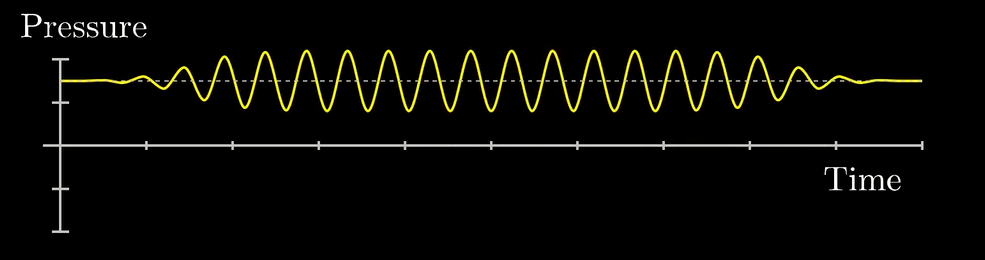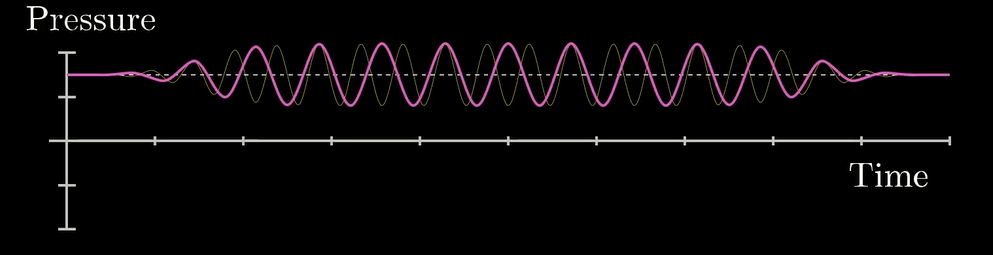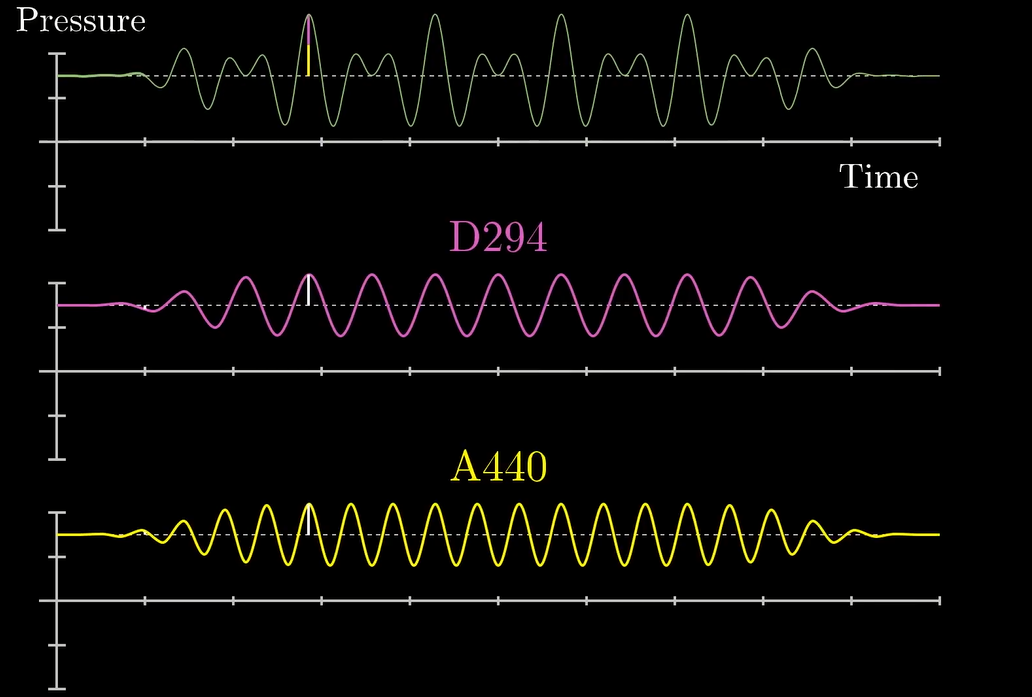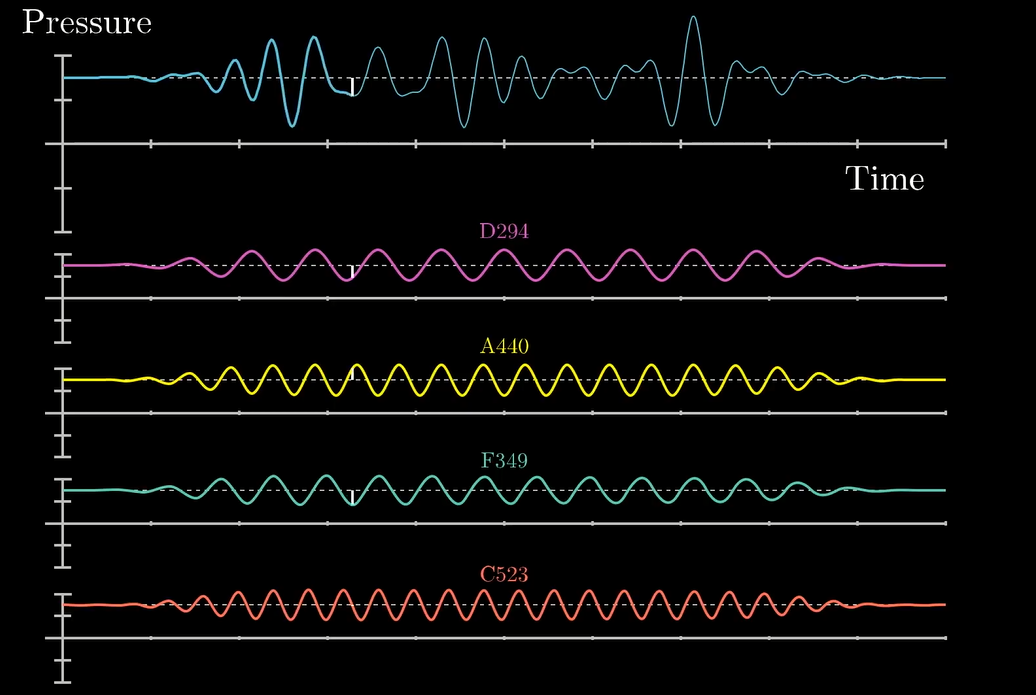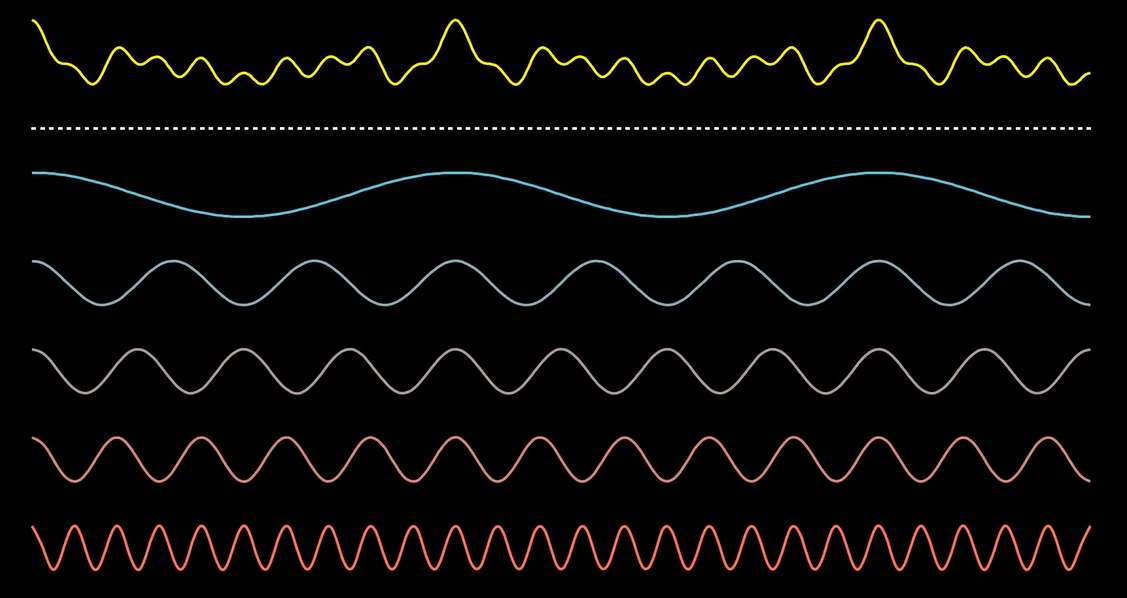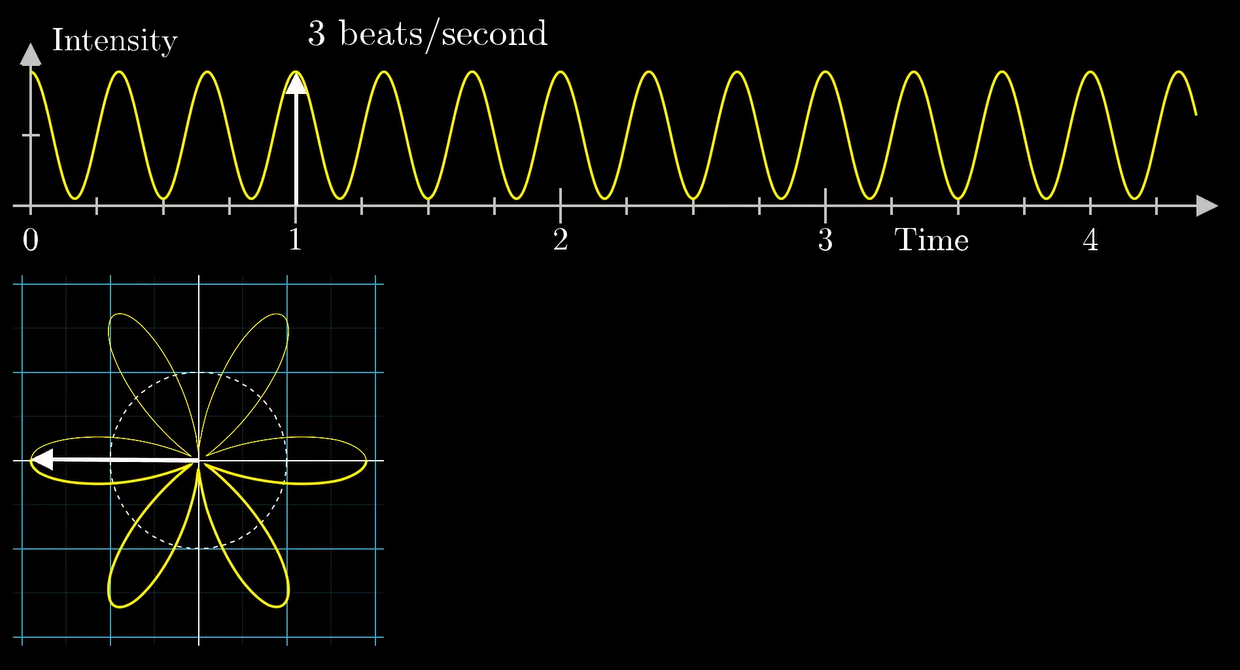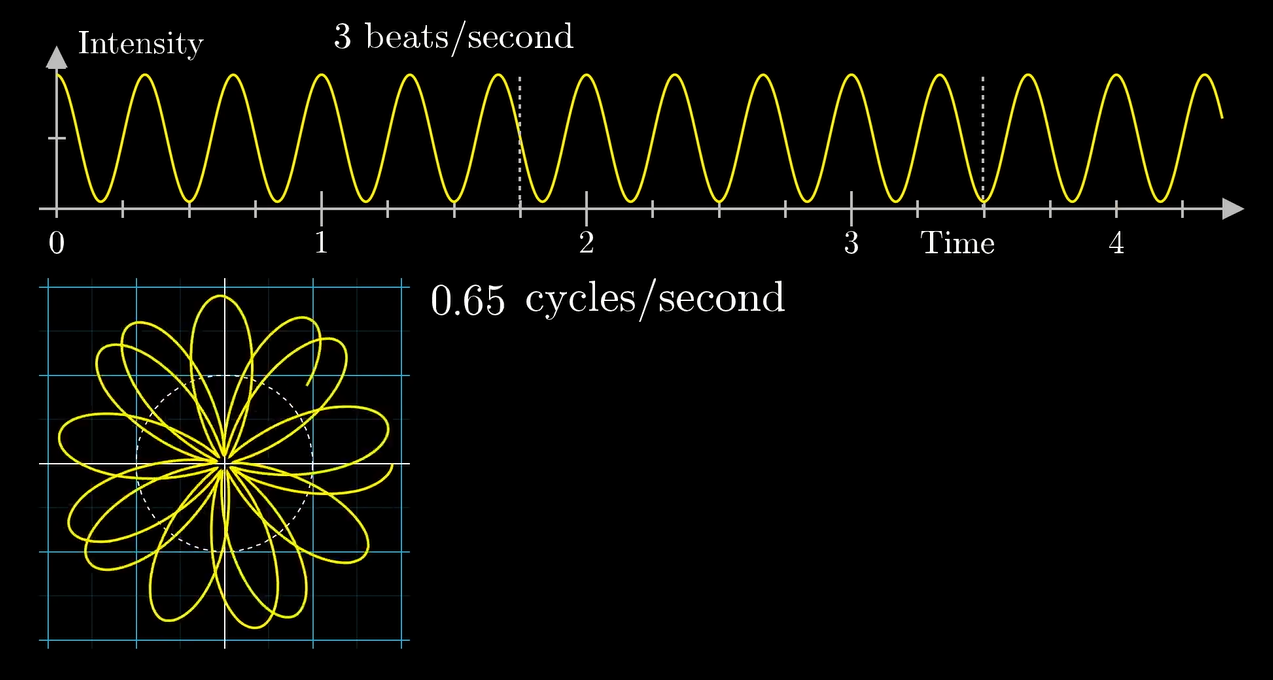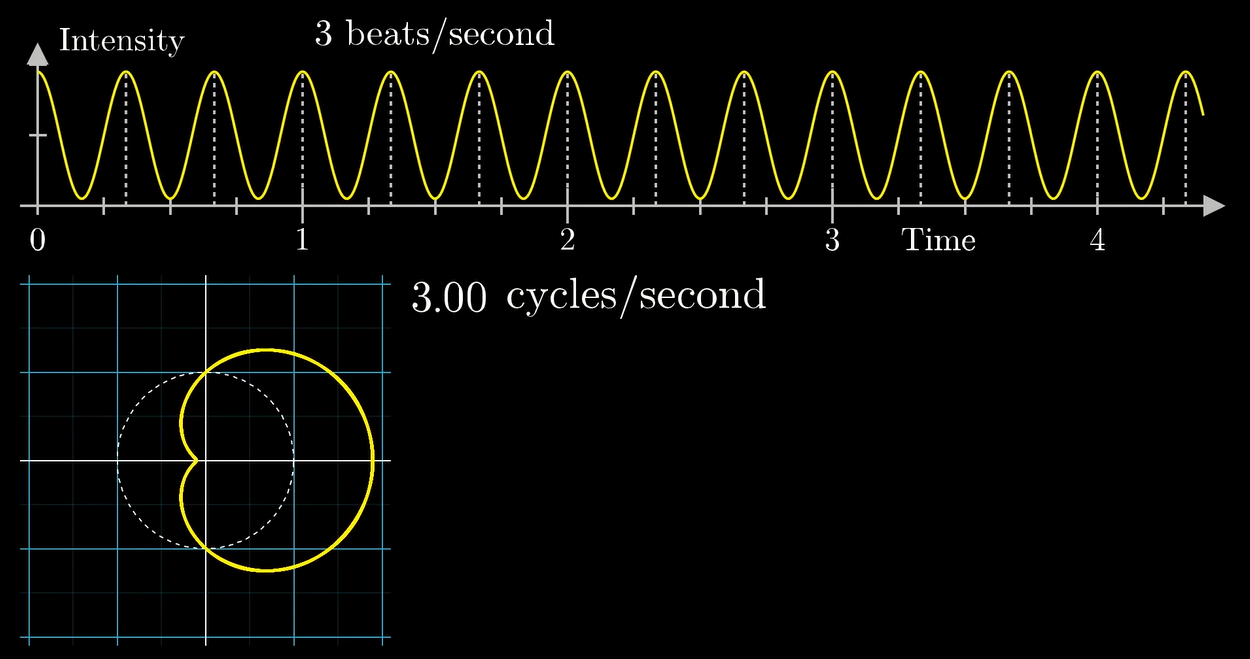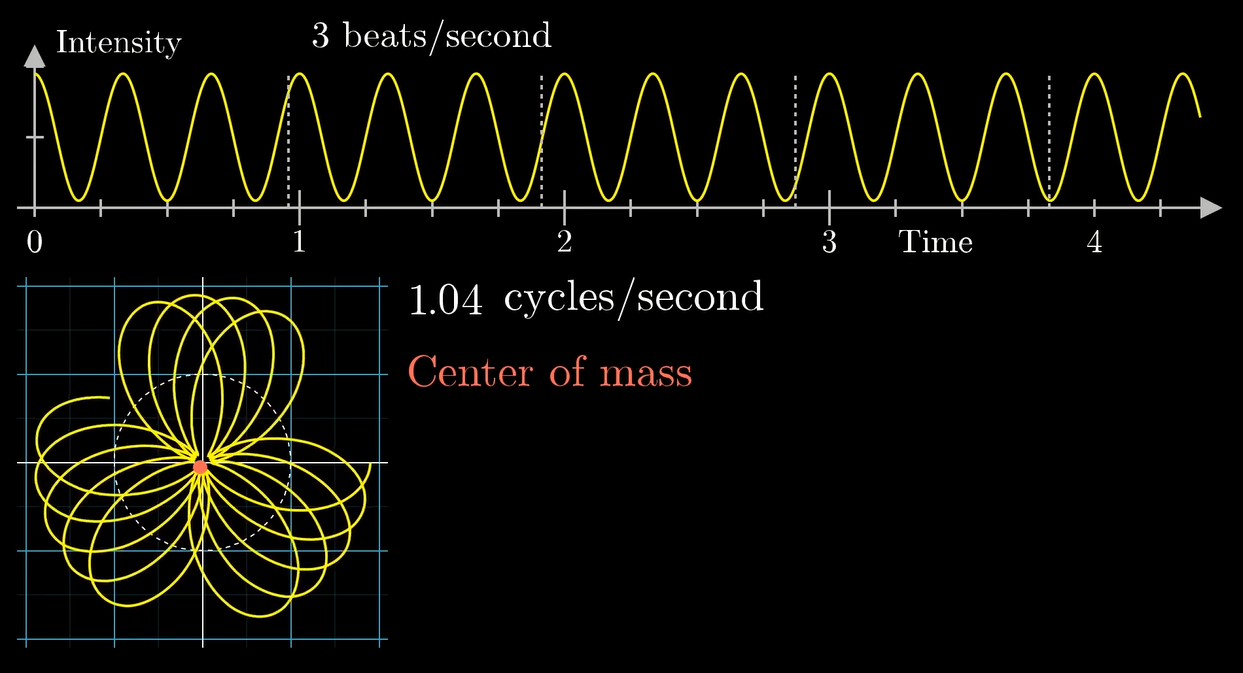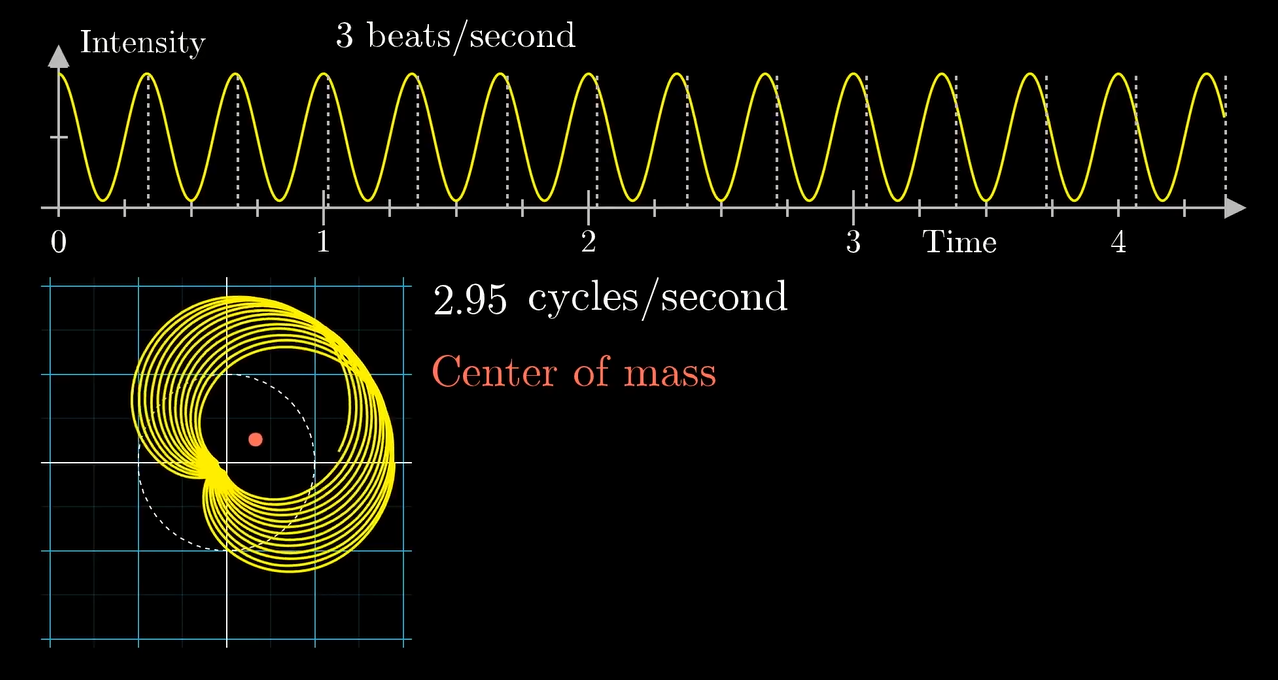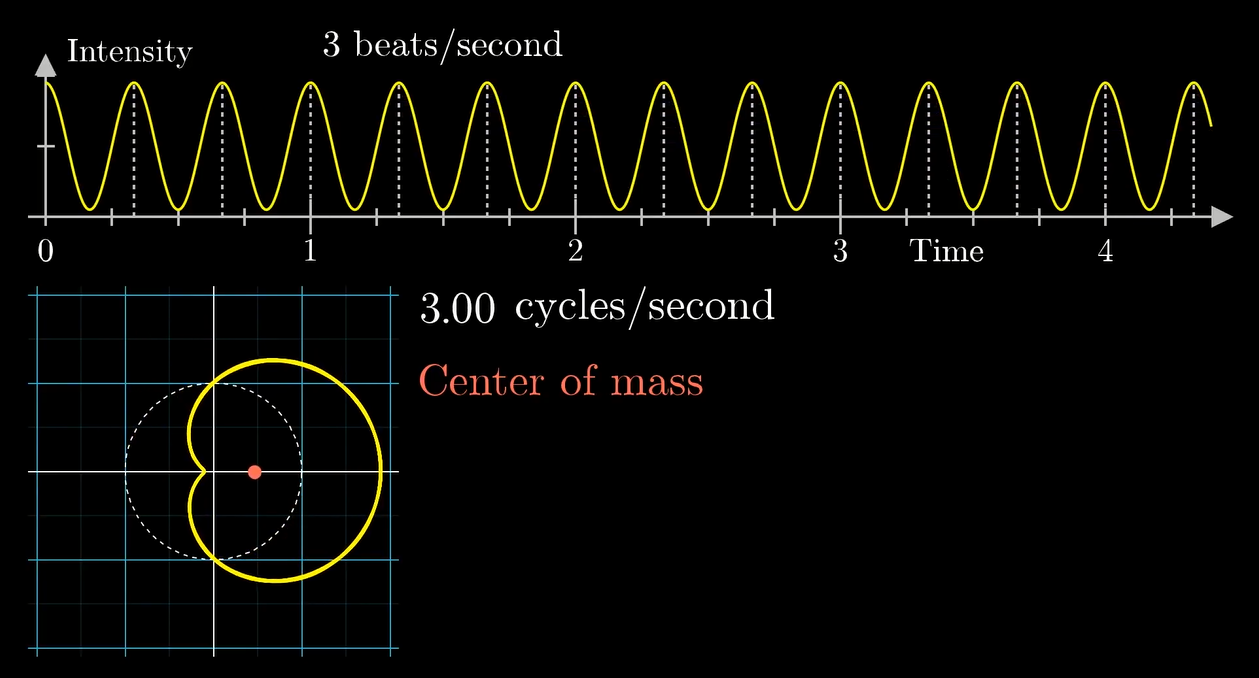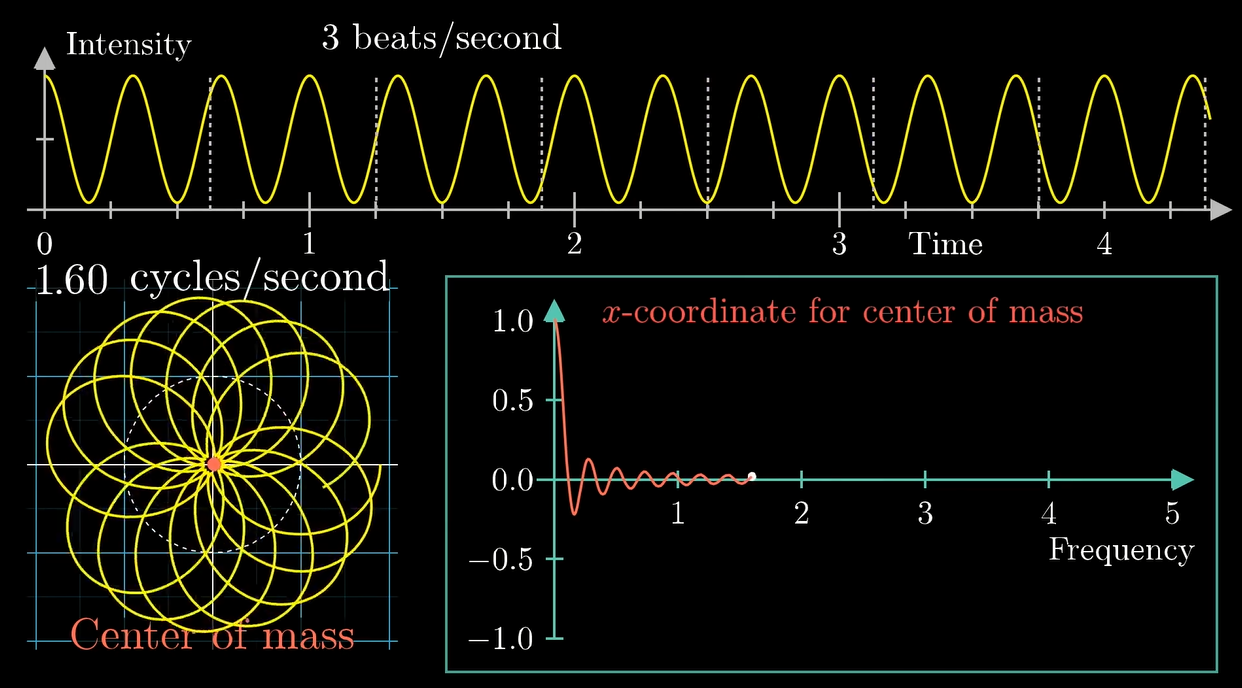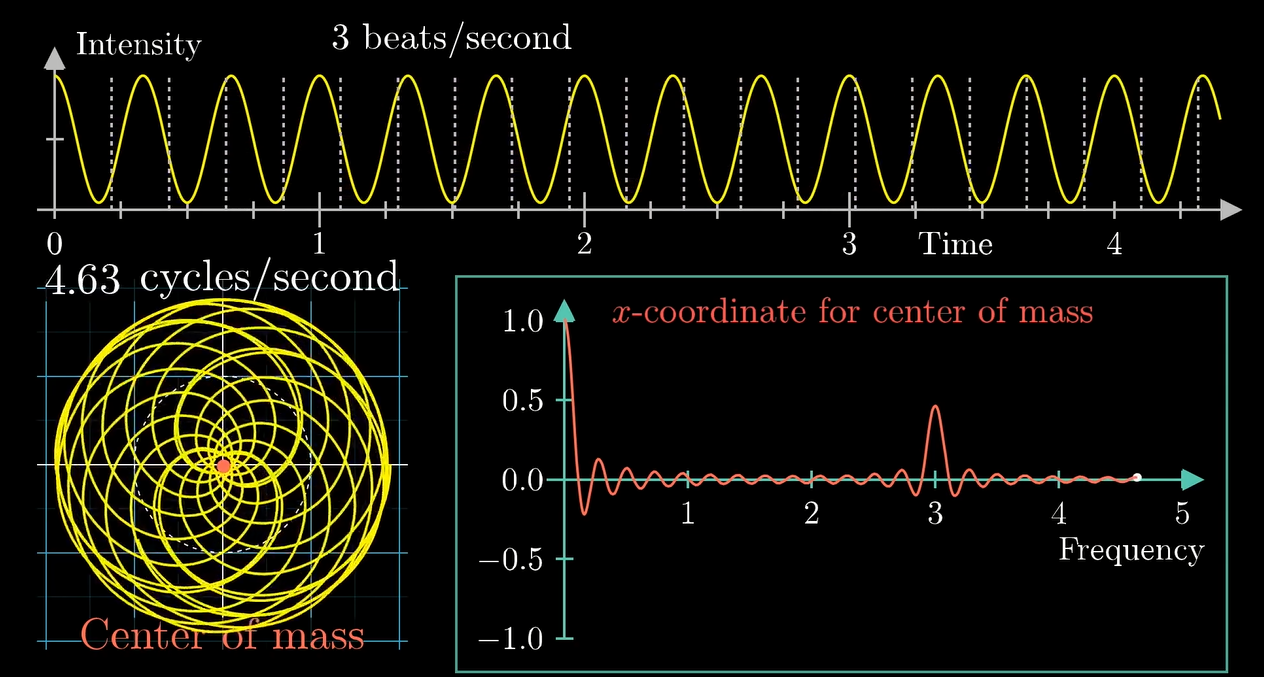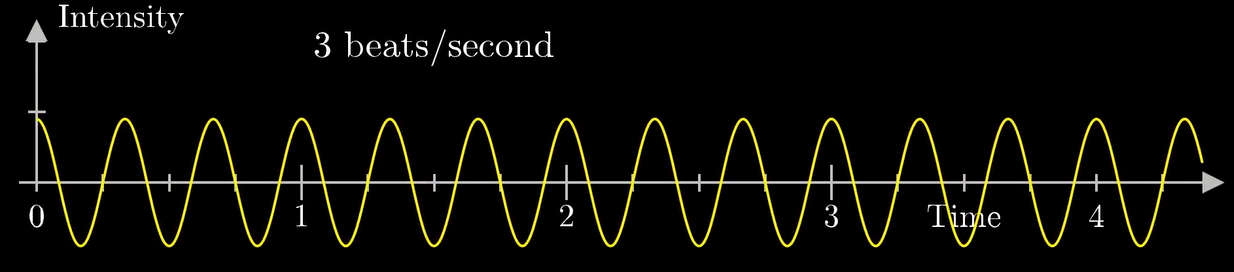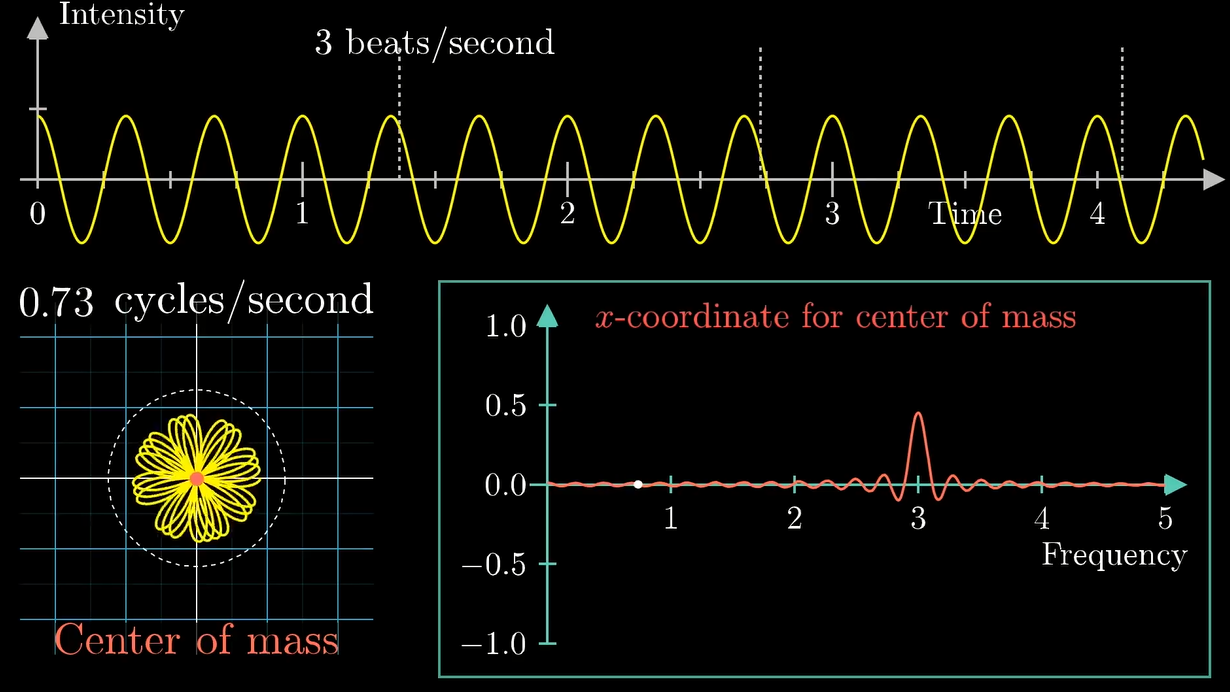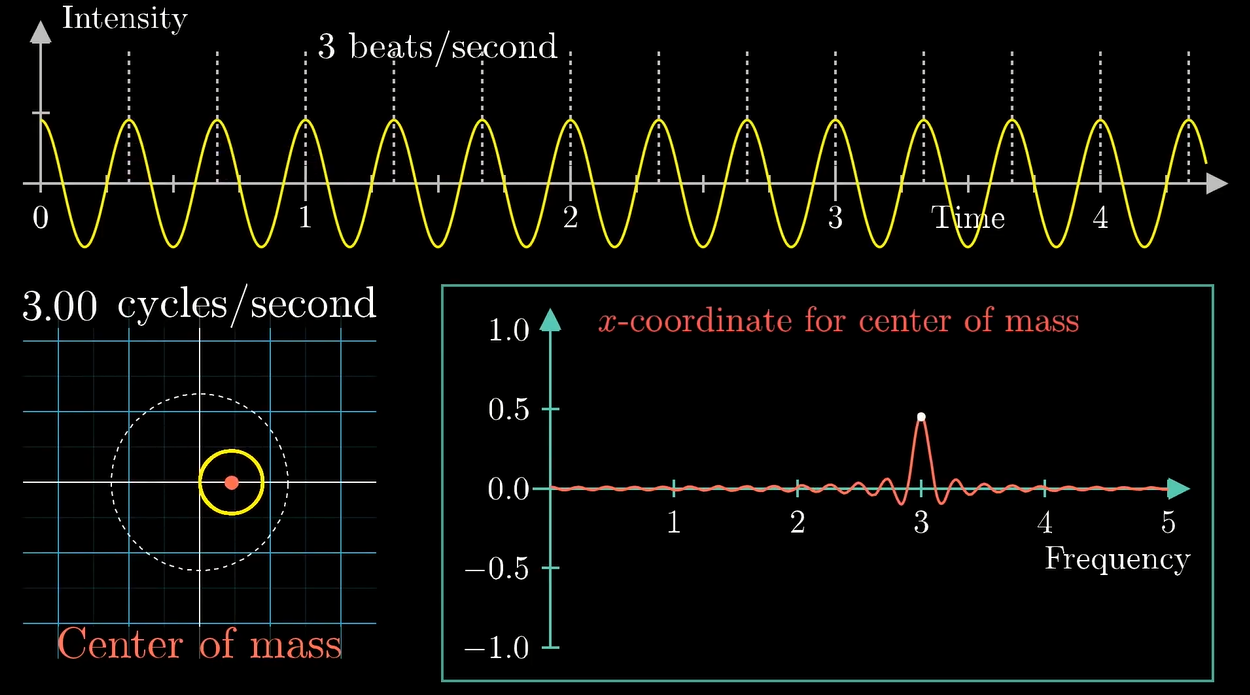(缠出来其实就是个圆, 因为波谷是负的)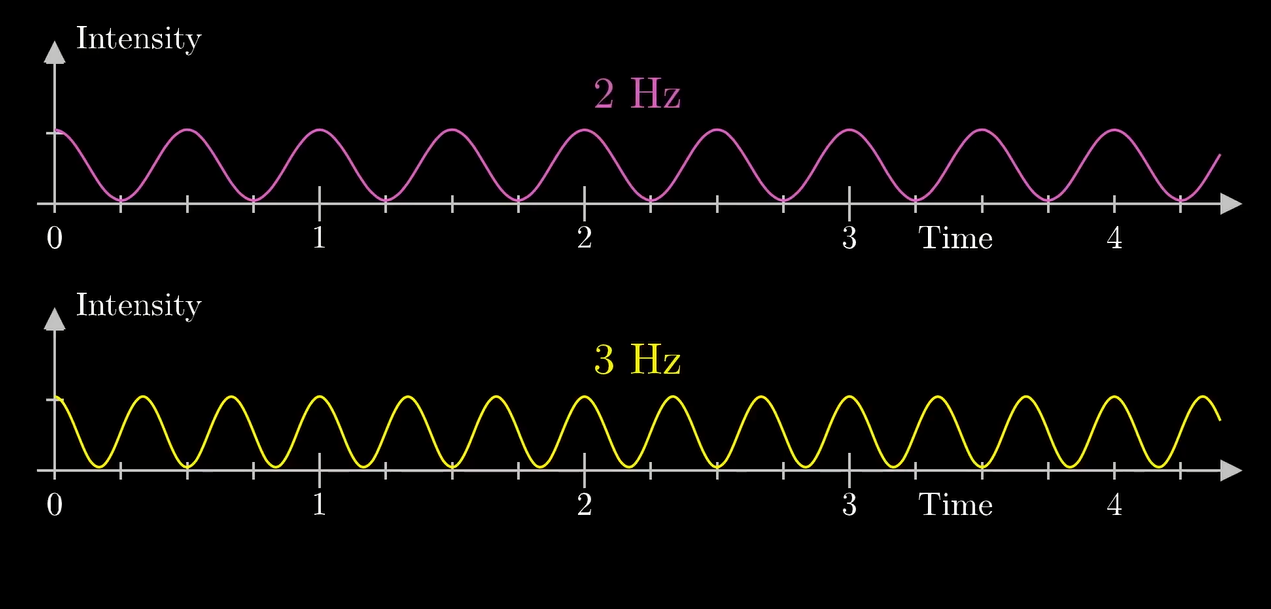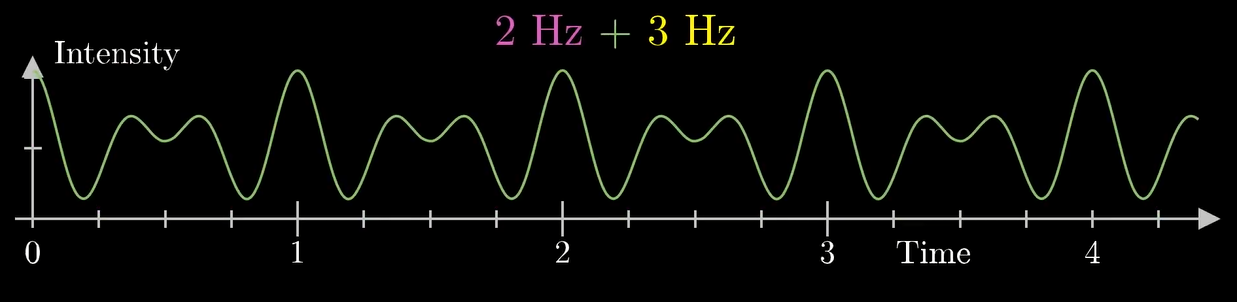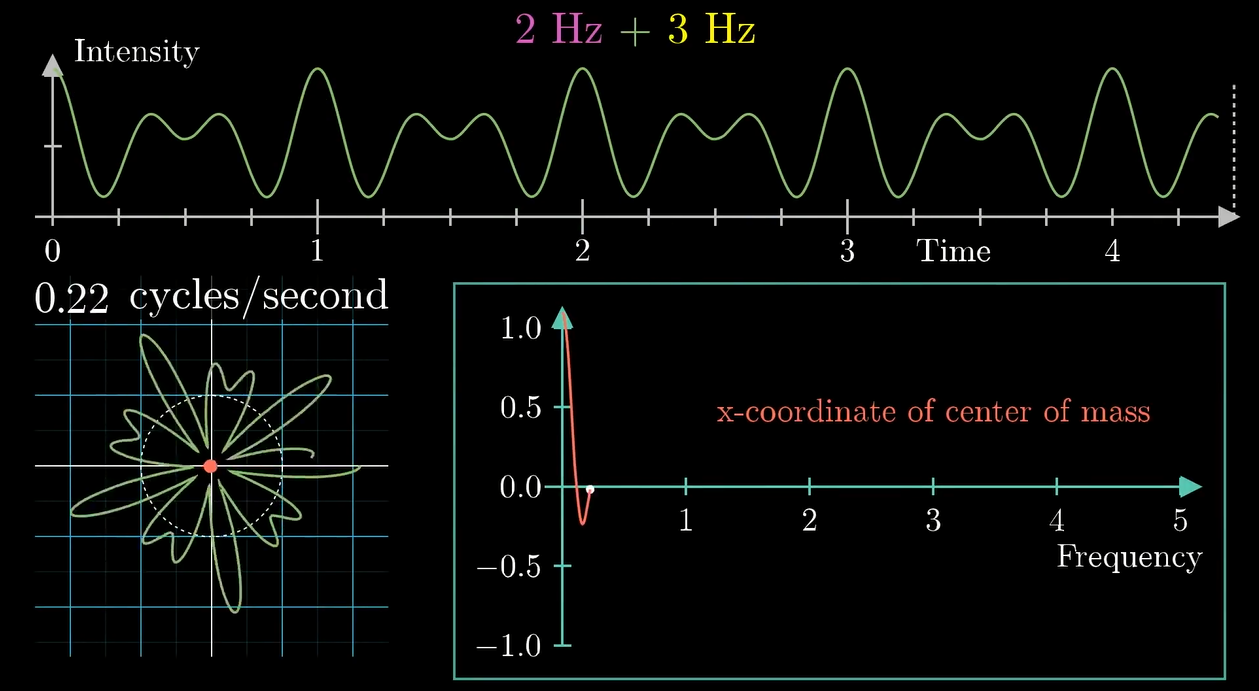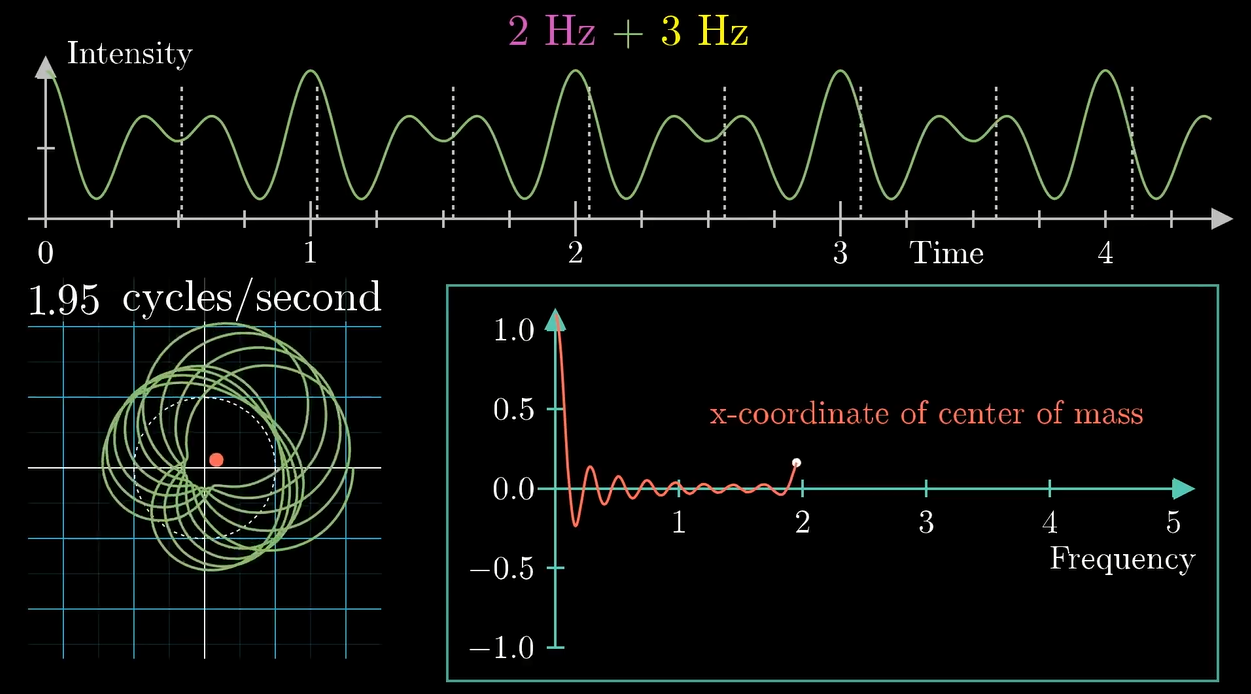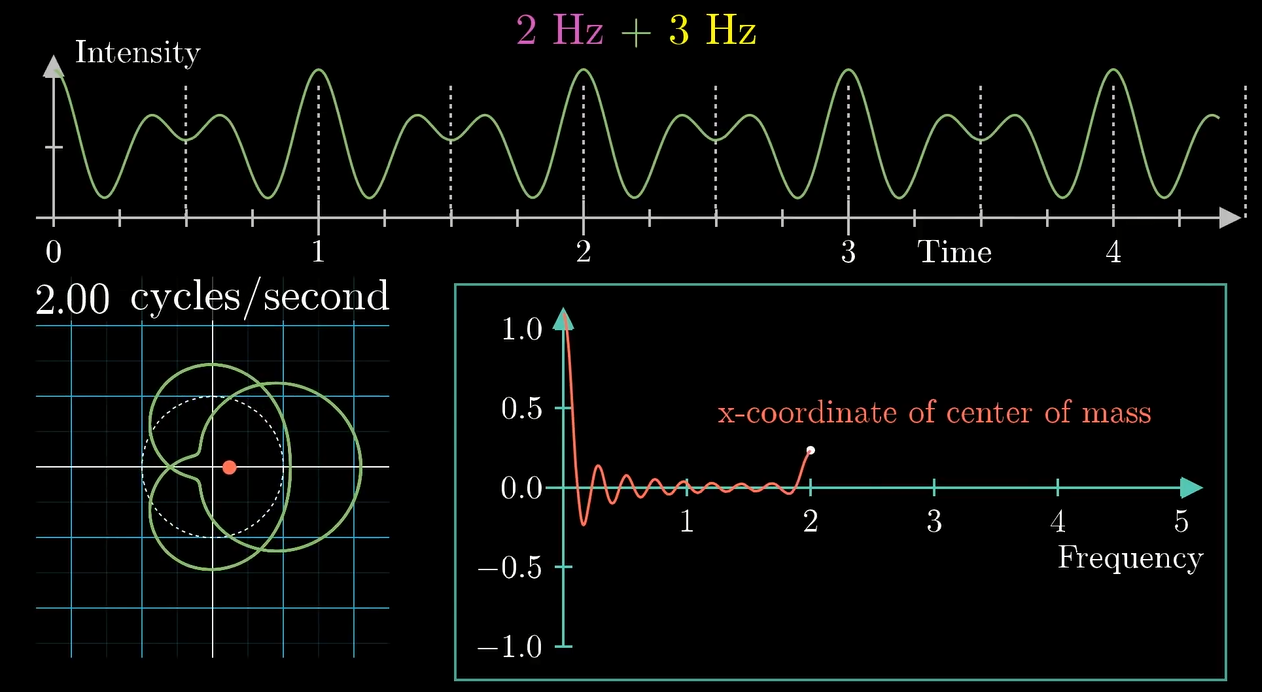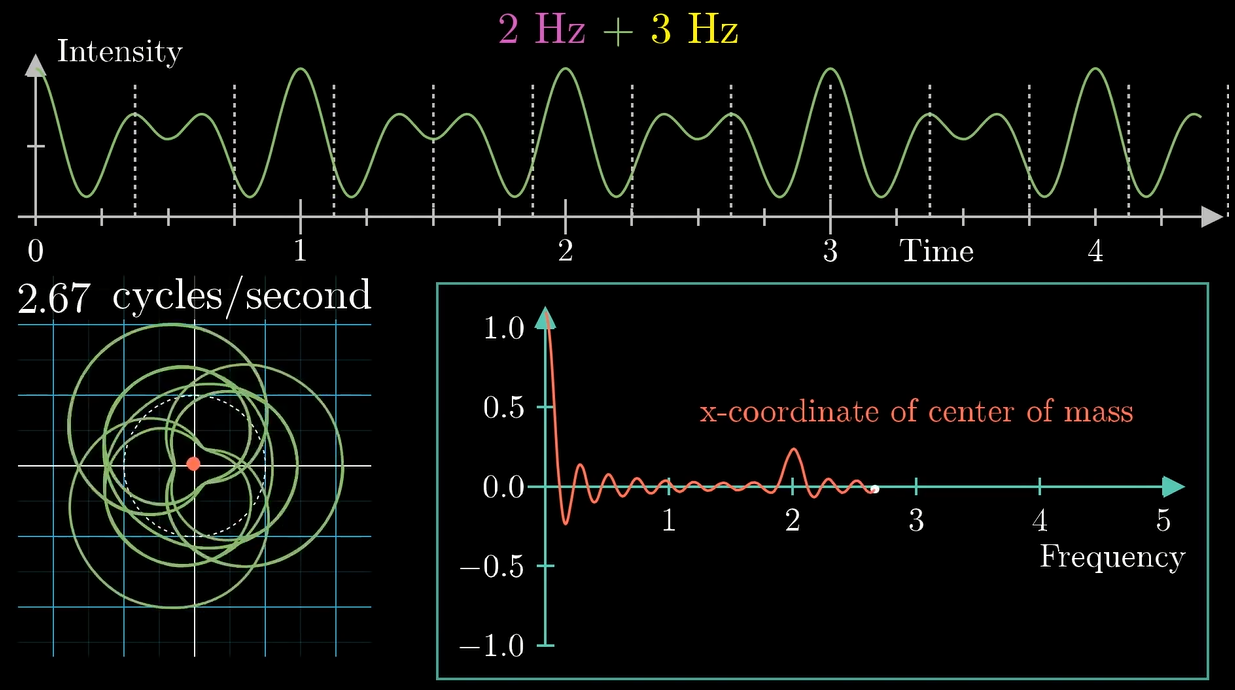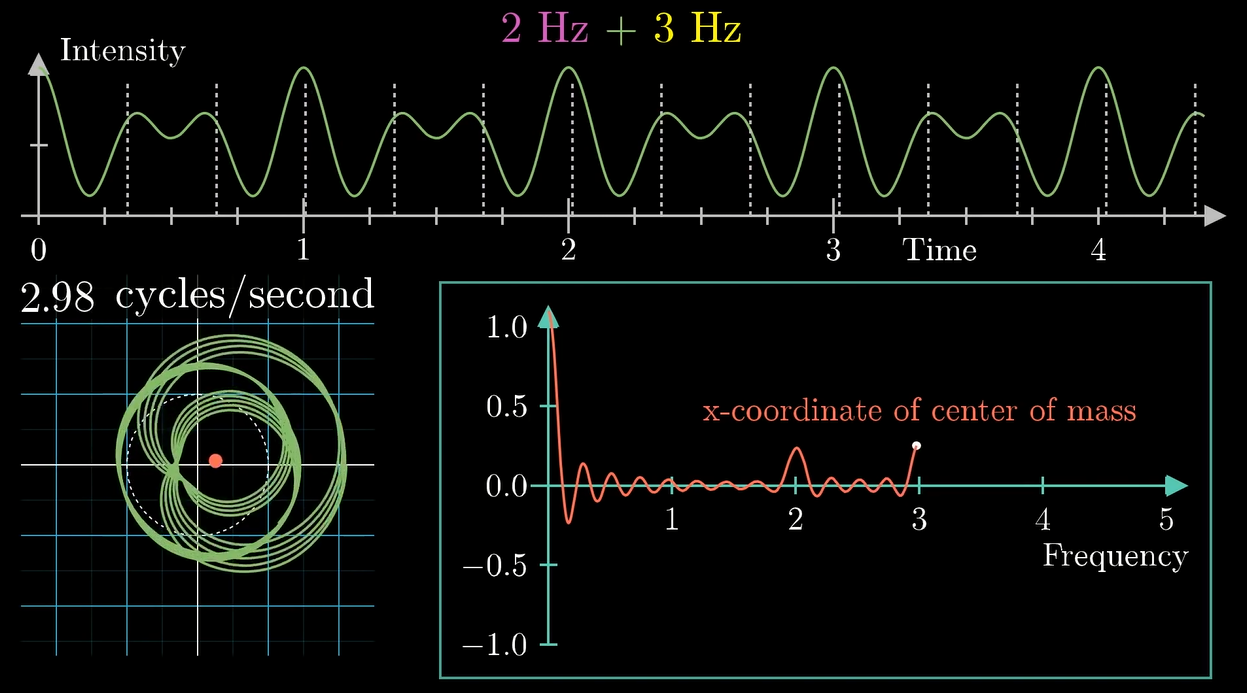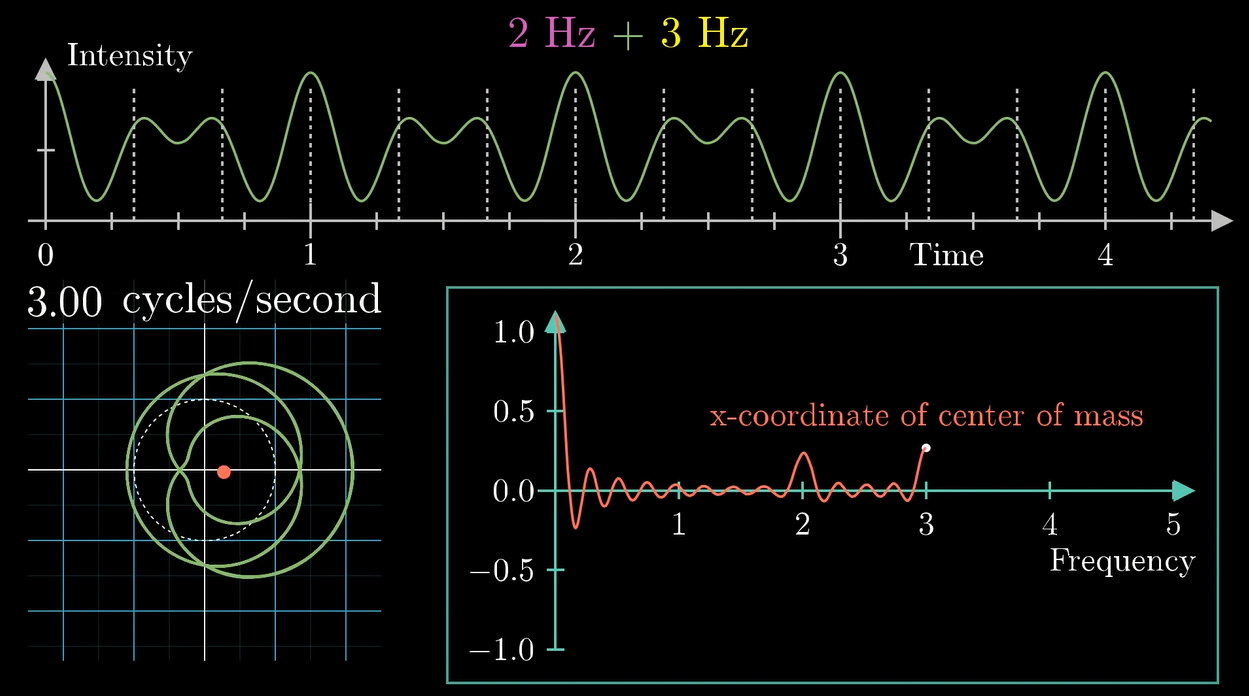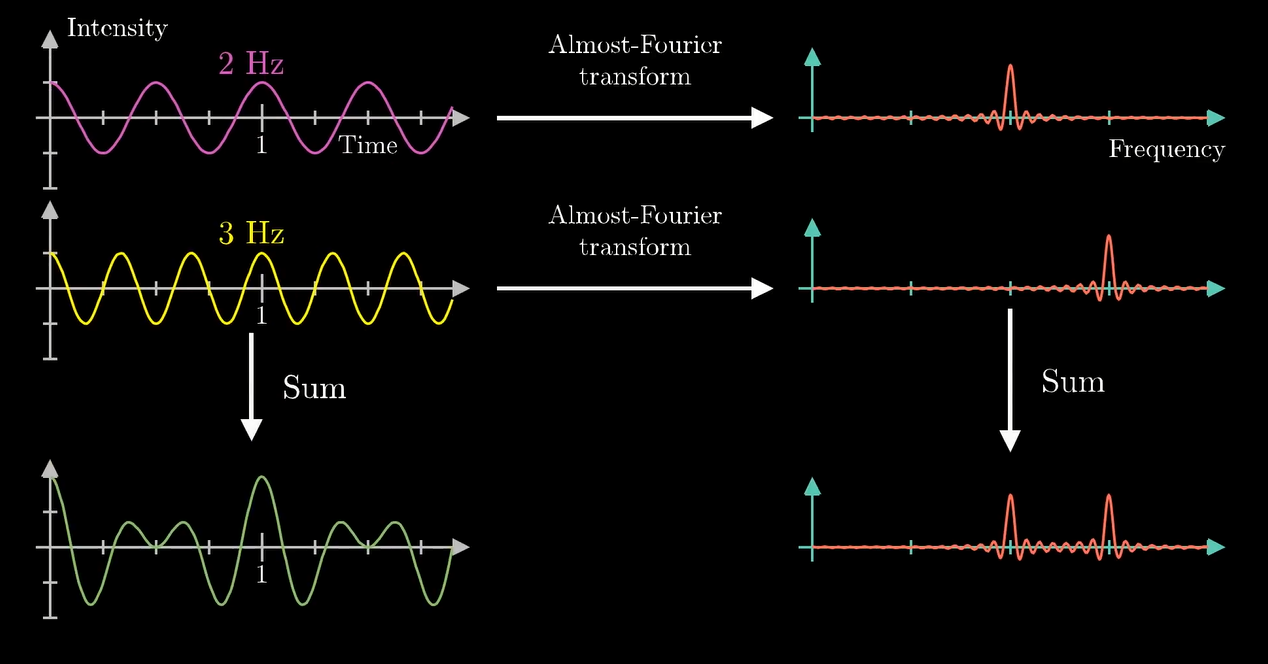### 变换的基础: 复数

$x^2=-1$

$i^2=-1$

$$i$$ 被称为虚数单位. 因为在创造它的时候认为它是个在自然界里没卵用的假数, 所以叫虚数.

(实际上物理里交流电分析里要用虚数的. 而且有一部分本来能做功的能量还会被虚部吃掉(变焦耳热了))

#### 复数的普通运算

$(a+bi)+(c+di)=(a+c)+(b+d)i \\ (a+bi)-(c+di)=(a-c)+(b-d)i$

\begin{align} (a+bi)(c+di)&=ac+a(di)+(bi)c+(bi)(di) \\ &=ac+adi+bci+bdi^2\\ &=ac+adi+bci-bd\\ &=(ac-bd)+(ad+bc)i \end{align}

$(r\angle\theta)(s\angle\varphi)=(rs)\angle(\theta+\varphi)$

$$\alpha$$ 为复数 $$a+bi$$ 的幅角, $$\beta$$ 为复数 $$c+bi$$ 的幅角, 则:

$\tan\alpha=\frac b a, \tan\beta=\frac d c$

\begin{align} \tan(\alpha+\beta)&=\frac{\tan\alpha + \tan\beta}{1-\tan\alpha\tan\beta} \\ &=\frac{\frac b a+\frac d c}{1-\frac{bd}{ac}} \\ &=\frac{\frac {bc} {ac}+\frac {ad} {ac}}{1-\frac{bd}{ac}} \\ &=\frac{bc+ad}{ac-bd} \end{align}

$$(a+bi)(c+di)$$ 就等于 $$(ac-bd)+(ad+bc)i$$.

(其实用 $$\tan$$ 来证明是不够严谨的, 因为它的周期是 $$\pi$$ 而不是 $$2\pi$$ ...然而 $$\sin$$$$\cos$$ 的式子太大懒得搞了...有兴趣自己试试吧)

\begin{aligned} \sqrt{a^2+b^2}\times\sqrt{c^2+d^2}&=\sqrt{(a^2+b^2)(c^2+d^2)} \\ &=\sqrt{a^2c^2+a^2d^2+b^2c^2+b^2d^2}\\ \sqrt{(ac-bd)^2+(ad+bc)^2}&=\sqrt{(a^2c^2-2abcd+b^2d^2)+(a^2d^2+2abcd+b^2c^2)}\\ &=\sqrt{a^2c^2+b^2d^2+a^2d^2+b^2c^2}\\ \\ \sqrt{a^2+b^2}\times\sqrt{c^2+d^2}&=\sqrt{(ac-bd)^2+(ad+bc)^2} \end{aligned}

#### 复数的高端运算

$e^{i\pi}+1=0$

$e^{i\varphi}=\cos\varphi+i\sin\varphi=1\angle\varphi$

$$\varphi$$$$\pi$$ 的时候就是欧拉公式辣 (815班旗上的元素之一)### 傅里叶变换

$F(t)=g(t)e^{2\pi ift}$

$F(t)=g(t)e^{-2\pi ift}$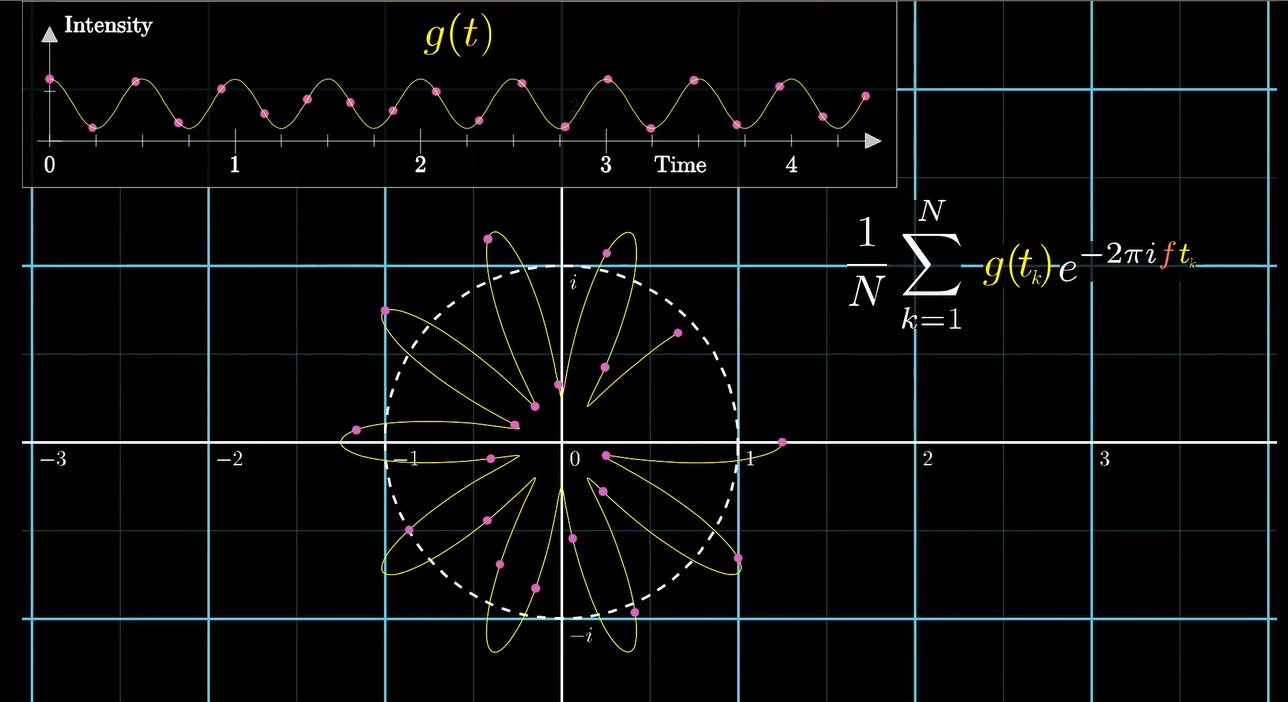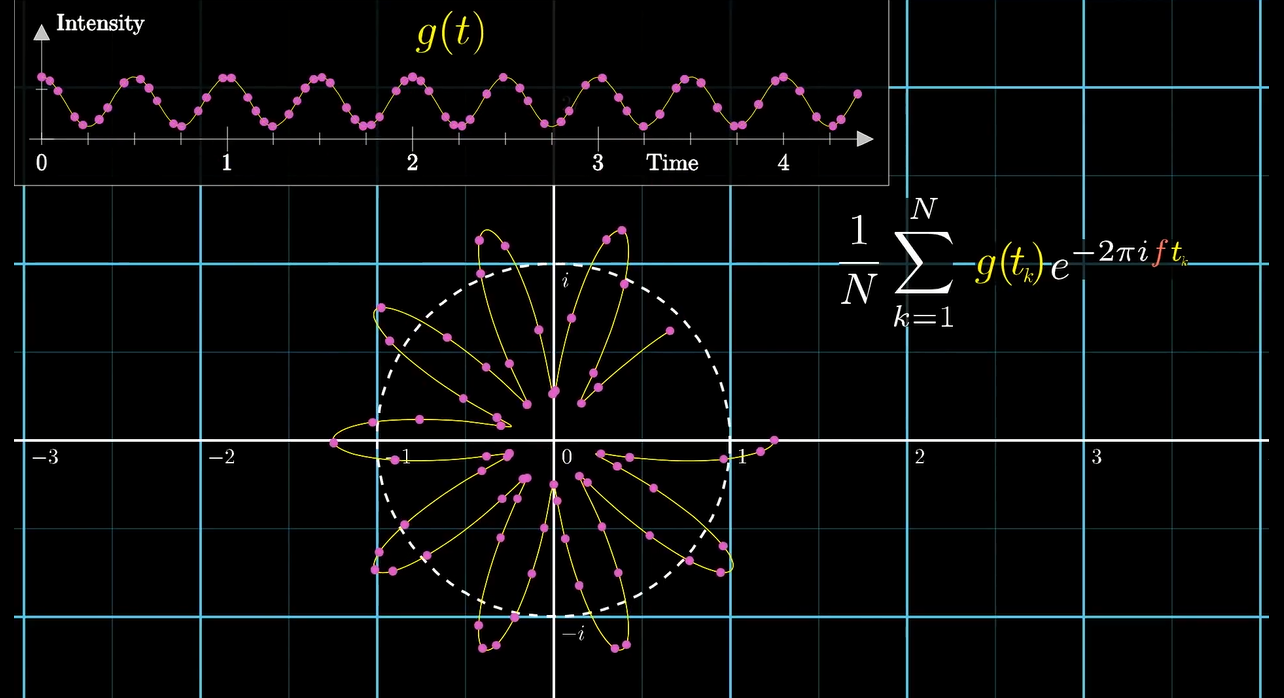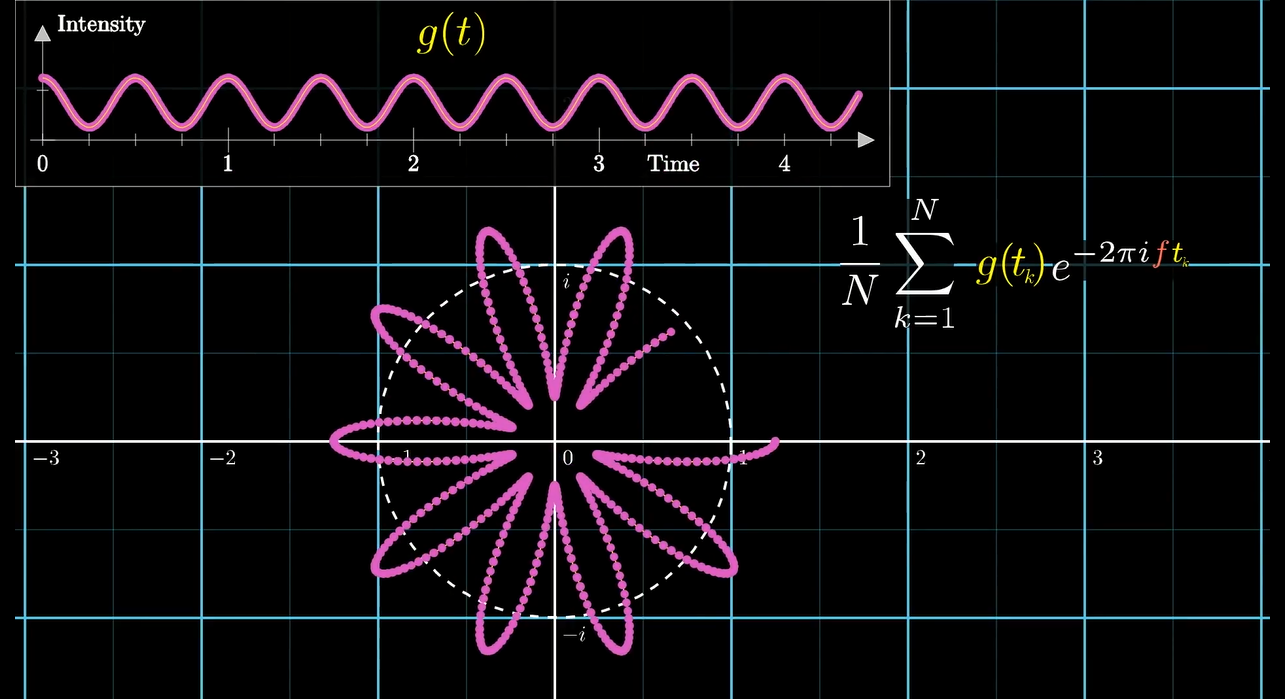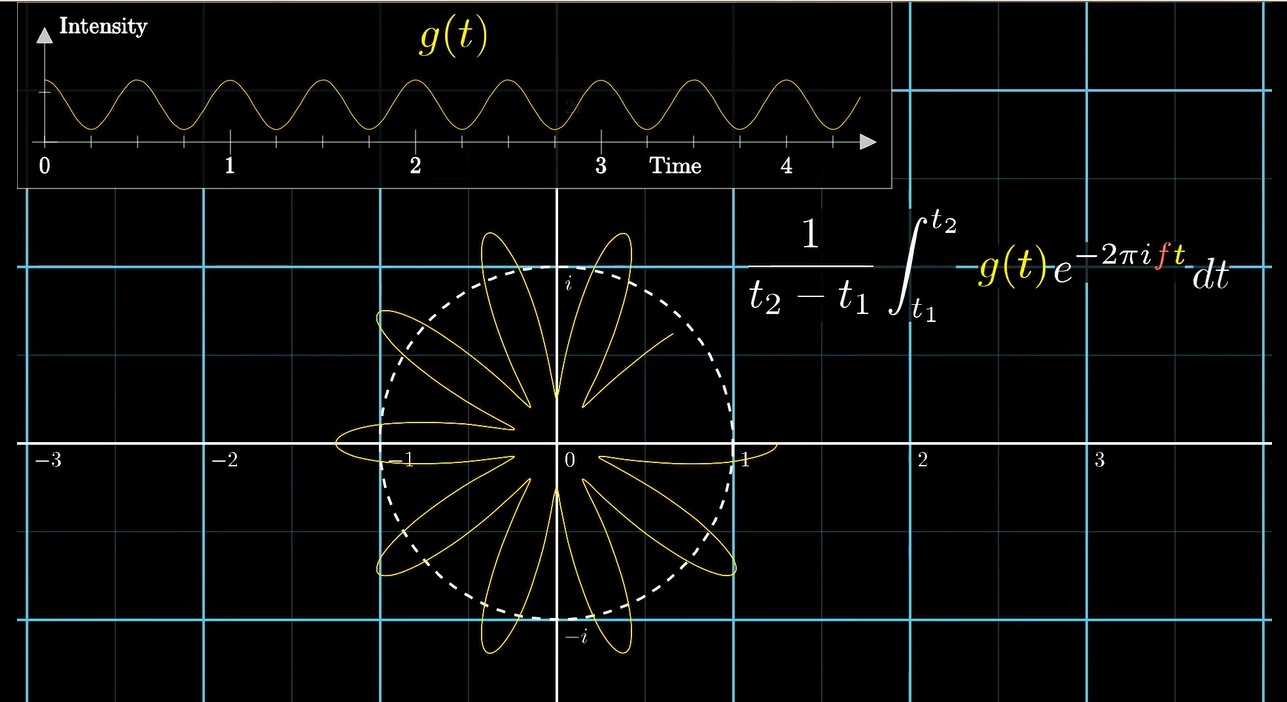$\frac 1 {t_2-t_1}\int_{t_1}^{t_2}g(t)e^{-2\pi ift}\text{d}t$

$\mathcal{F}(f)=\int_{-\infty}^{\infty}g(t)e^{-2\pi ift}\text{d}t$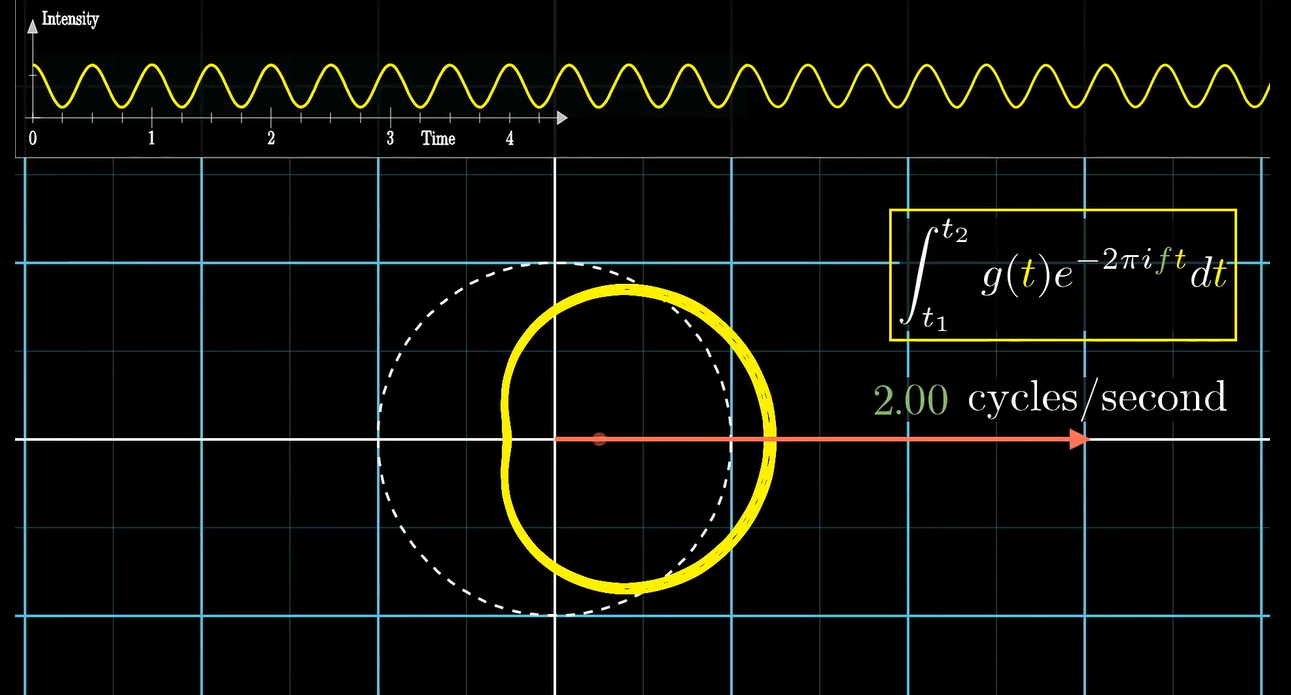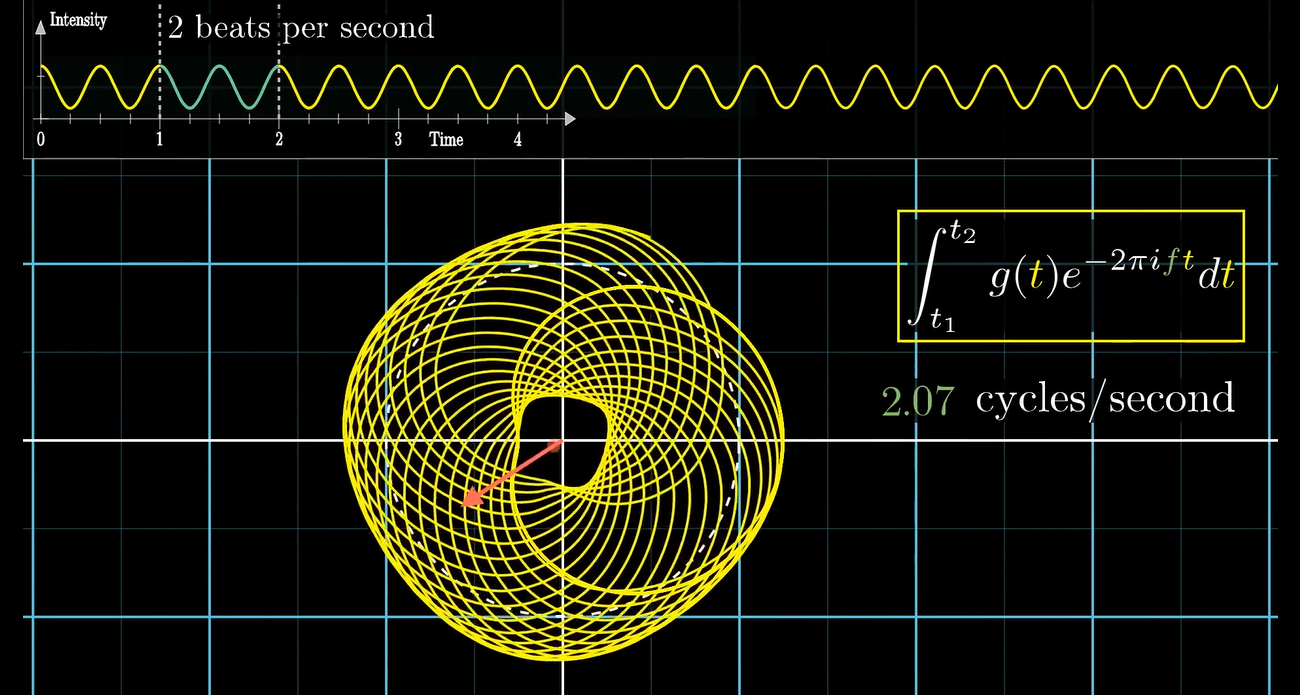### 离散傅里叶变换$\mathcal{F}(f)=\sum_{k=1}^Ng(t_k)e^{-2\pi i f t_k}$

## FFT 与多项式

$f(x)=\sum_{k=0}^{N-1}g(k)\omega_n^{kx}$

### $$n$$ 次单位复根

$$n$$ 次单位复根是满足 $$\omega^n=1$$ 的复数 $$\omega$$. 根据复数乘法的集合意义以及同余, 我们可以得出: $$n$$ 次单位根恰好有 $$n$$ 个, 均匀分布在以原点为圆心, 半径为 $$1$$ 的圆周上. 如下图所示: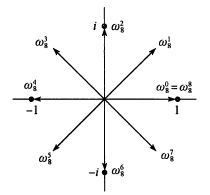$\omega_n^n=\omega_n^0=1$

$a\equiv b \pmod n \Rightarrow \omega_n^a=\omega_n^b$

### 消去引理, 折半引理与求和引理

$\omega_{dn}^{dk}=\omega_n^k$

$\omega_{dn}^{dk}=(e^{2\pi i / dn})^{dk}=(e^{2\pi i /n})^k= \omega_n^k$

$(\omega_n^{k+n/2})^2=\omega_n^{2k+n}=\omega_n^{2k}\omega_n^n=\omega_n^{2k}=(\omega_n^k)^2$

$$\blacksquare$$

$\sum_{j=0}^{n-1}(\omega_n^k)^j=0$

$\sum_{k=0}^nx^k=\frac{x^{n+1}-1}{x-1}$

$\sum_{j=0}^{n-1}(\omega_n^k)^j=\frac{(\omega_n^k)^n-1}{\omega_n^k-1}=\frac{(\omega_n^n)^k-1}{\omega_n^k-1}=\frac{(1)^k-1}{\omega_n^k-1}=0$

$$\blacksquare$$

### 重新定义

$F(x)=\sum_{k=0}^{N-1}g(k)x^k$

$$f(x)$$ 就成了:

$f(x)=F(\omega_n^x)$

### 多项式的表示

#### 多项式的系数表达

$A(x) = \sum _{i=0} ^{n-1} a_i x^i$

$A(x_0) = a_0+x_0(a_1+x_0(a_2+...+x_0(a_{n-2}+x_0 a_{n-1})...))$

$c_i=\sum_{j=0}^i a_jb_{i-j}$

#### 多项式的点值表达

$\{(x_0,y_0),(x_1,y_1),(x_2,y_2),...,(x_{n-1},y_{n-1})\}$

(我们可以先暂且把 $$A(x)$$ 看作一个函数)

....

#### 求值与插值

$\begin{bmatrix} 1 & x_0 & x_0^2 & \dots & x_0^{n-1} \\ 1 & x_1 & x_1^2 & \dots & x_1^{n-1} \\ \vdots & \vdots & \vdots & \ddots & \vdots \\ 1 & x_{n-1} & x_{n-1}^2 & \dots & x_{n-1}^{n-1} \end{bmatrix} \begin{bmatrix} a_0 \\ a_1 \\ \vdots \\ a_{n-1} \end{bmatrix} = \begin{bmatrix} y_0 \\ y_1 \\ \vdots \\ y_{n-1} \end{bmatrix}$

$\prod _{0\leq j < k \leq n-1} (x_k-x_j)$

$\vec{a}=V(x_0,x_1,...,x_{n-1})^{-1}\vec{y}$

$$\blacksquare$$

$A(x)=\sum_{k=0}^{n-1} y_k \frac{\prod _{j\neq k} (x-x_j)} {\prod_{j\neq k} (x_k-x_j)}$

### 快速傅里叶变换FFT

FFT (Fast Fourier Transform)利用了 $$n$$ 次单位根的特殊性质, 让我们可以在 $$O(n\log n)$$ 的时间内计算出DFT.

​ 我们下面统一这样定义DFT:

​ 对于 $$A$$ 的系数表达 $$\vec{a}= (a_0,a_1,...,a_{n-1})$$$$k=0,1,...,n-1$$ , 定义结果 $$y_k$$:

$y_k=A(\omega_n^k)=\sum_{i=0}^{n-1}a_i\omega^{ki}_n$

​ 则 $$\vec{y}=(y_0,y_1,...,y_{n-1})$$ 就是系数向量 $$\vec{a}$$离散傅里叶变换(DFT). 简记为 $$\vec{y}=DFT_n(\vec{a})$$

FFT使用的是分治策略. 根据系数下标的奇偶来拆分子问题, 将这些系数分配到两个次数界为 $$n/2$$ 的多项式 $$A^{}(x)$$$$A^{}(x)$$ :

$A^{}(x)=a_0+a_2x+a_4x^2+...+a_{n-2}x^{n/2-1}$

$A^{}(x)=a_1+a_3x+a_5x^2+...+a_{n-1}x^{n/2-1}$

$A(x)=A^{}(x^2)+A^{}(x^2)x$

1.求多项式 $$A^{}(x)$$ 和 多项式 $$A^{}(x)$$ 在下列点上的取值:

$(\omega_n^0)^2,(\omega_n^1)^2,...(\omega_n^{n-1})^2$

2.根据刚刚推导出的将两个按奇偶拆开的多项式的值合并的公式合并结果.

void FFT(Complex* a,int len){
if(len==1)
return;
Complex* a0=new Complex[len/2];
Complex* a1=new Complex[len/2];
for(int i=0;i<len;i+=2){
a0[i/2]=a[i];
a1[i/2]=a[i+1];
}
FFT(a0,len/2);
FFT(a1,len/2);
Complex wn(cos(2*Pi/len),sin(2*Pi/len));
Complex w(1,0);
for(int i=0;i<(len/2);i++){
a[i]=a0[i]+w*a1[i];
a[i+len/2]=a0[i]-w*a1[i];
w=w*wn;
}
delete[] a0;
delete[] a1;
}


$y_0=a_0\omega_1^0=a_0$

$y_k^{}=A^{}(\omega_{n/2}^k)$

$y_k^{}=A^{}(\omega_{n/2}^k)$

$y_k^{}=A^{}(\omega_n^{2k})$

$y_k^{}=A^{}(\omega_n^{2k})$

\begin{aligned} y_k&=y^{}_k+\omega_n^ky_k^{} \\ &=A^{}(\omega_n^{2k})+\omega_n^kA^{}(\omega_n^{2k}) \\ &= A(\omega_n^k) \end{aligned}

\begin{aligned} y_{k+(n/2)} &= y^{}_{k+(n/2)} - \omega_n^ky_{k+(n/2)}^{} \\ &= y_k^{} + \omega_n^{k+(n/2)} y_k^{} \\ &= A^{}(\omega_n^{2k}) +\omega_n^{k+(n/2)} A^{}(\omega_n^{2k}) \\ &= A^{}(\omega_n^{2k+n}) + \omega_n^{k+(n/2)} A^{}(\omega_n^{2k+n}) \\ &= A(\omega_n^{k+(n/2)}) \end{aligned}

$T(n)=2T(n/2)+\Theta(n)=\Theta(n\log(n))$

### 通过 FFT 在单位复数根处插值

$\begin{bmatrix} y_0 \\ y_1 \\ y_2 \\ y_3 \\ \vdots \\ y_{n-1} \end{bmatrix}= \begin{bmatrix} 1 & 1 & 1 & 1 & \dots & 1 \\ 1 & \omega_n & \omega_n^2 & \omega_n^ 3 & \dots & \omega_n^{n-1} \\ 1 & \omega_n^2 & \omega_n^4 & \omega_n^6 & \dots & \omega_n^{2(n-1)} \\ 1 & \omega_n^3 & \omega_n^6 & \omega_n^9 & \dots & \omega_n^{3(n-1)} \\ \vdots & \vdots & \vdots & \vdots & \ddots & \vdots \\ 1 & \omega_n^{n-1} & \omega_n^{2(n-1)} & \omega_n^{3(n-1)} & \dots & \omega_n^{(n-1)(n-1)} \end{bmatrix} \begin{bmatrix} a_0 \\ a_1 \\ a_2 \\ a_3 \\ \vdots \\ a_{n-1} \end{bmatrix}$

$[V_n^{-1}V_n]_{jj'}=\sum_{k=0}^{n-1}(\omega_n^{-kj}/n)(\omega_n^{kj'})=\sum_{k=0}^{n-1}\omega_n^{k(j'-j)}/n$

$$j'=j$$ 时, 此值为 $$1$$ , 否则根据求和引理, 此和为 $$0$$.

$$\blacksquare$$

$a_j=\frac{1}{n} \sum_{k=0}^{n-1} y_k\omega_n^{-kj}$

void FFT(Complex* a,int len,int opt){
if(len==1)
return;
Complex* a0=new Complex[len/2];
Complex* a1=new Complex[len/2];
for(int i=0;i<len;i+=2){
a0[i/2]=a[i];
a1[i/2]=a[i+1];
}
FFT(a0,len/2);
FFT(a1,len/2);
Complex wn(cos(2*Pi/len),opt*sin(2*Pi/len));
Complex w(1,0);
for(int i=0;i<(len/2);i++){
a[i]=a0[i]+w*a1[i];
a[i+len/2]=a0[i]-w*a1[i];
w=w*wn;
}
delete[] a0;
delete[] a1;
}


### FFT的速度优化与迭代实现

for(int i=0;i<(len/2);i++){
int t=w*a1[i];
a[i]=a0[i]+t;
a[i+len/2]=a0[i]-t;
w=w*wn;
}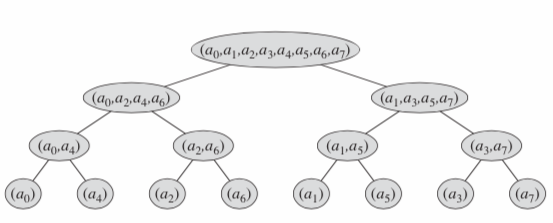(没错这图就是算导上的)

• 首先成对取出元素, 对于每对元素进行 $$1$$ 次蝴蝶操作计算出它们的 $$DFT$$ 并用它们的 $$DFT$$ 替换原来的两个元素, 这样 $$\vec a$$ 中就会存储有 $$n/2$$ 个二元素 $$DFT$$ ;

• 接着继续成对取出这 $$n/2$$$$DFT$$ , 对于每对 $$DFT$$ 进行 $$2$$ 次蝴蝶操作计算出包含 $$4$$ 个元素的 $$DFT$$ , 并用计算出的 $$DFT$$ 替换掉原来的值.

• 重复上面的步骤, 直到计算出 $$2$$ 个长度为 $$n/2$$$$DFT$$ , 最后使用 $$n/2$$ 次蝴蝶操作即可计算出整个向量的 $$DFT$$.

void FFT(Complex* a,int len,int opt){
for(int i=0;i<len;i++)
if(i<rev[i])
std::swap(a[i],a[rev[i]]);
for(int i=1;i<len;i<<=1){
Complex wn=Complex(cos(PI/i),opt*sin(PI/i));
int step=i<<1;
for(int j=0;j<len;j+=step){
Complex w=Complex(1,0);
for(int k=0;k<i;k++,w=w*wn){
Complex x=a[j+k];
Complex y=w*a[j+k+i];
a[j+k]=x+y;
a[j+k+i]=x-y;
}
}
}
}


for(int i=0;i<bln;i++)
rev[i]=(rev[i>>1]>>1)|((i&1)<<(bct-1));


#include <bits/stdc++.h>

const int MAXN=270000;
const int DFT=1;
const int IDFT=-1;
const double PI=acos(-1);

struct Complex{
double real;
double imag;
Complex(double r=0,double i=0){
this->real=r;
this->imag=i;
}
};
Complex operator+(const Complex& a,const Complex& b){
return Complex(a.real+b.real,a.imag+b.imag);
}
Complex operator-(const Complex& a,const Complex& b){
return Complex(a.real-b.real,a.imag-b.imag);
}
Complex operator*(const Complex& a,const Complex& b){
return Complex(a.real*b.real-a.imag*b.imag,a.real*b.imag+a.imag*b.real);
}

Complex a[MAXN],b[MAXN],c[MAXN];

int n;             //Length of a
int m;             //Length of b
int bln=1;         //Binary Length
int bct;           //Bit Count
int len;           //Length Sum
int rev[MAXN];     //Binary Reverse Sort

void Initialize();
void FFT(Complex*,int,int);

int main(){
Initialize();
FFT(a,bln,DFT);
FFT(b,bln,DFT);
for(int i=0;i<=bln;i++){
c[i]=a[i]*b[i];
}
FFT(c,bln,IDFT);
for(int i=0;i<=len;i++){
printf("%d ",int(c[i].real/bln+0.5));
}
putchar('\n');
return 0;
}

void FFT(Complex* a,int len,int opt){
for(int i=0;i<len;i++)
if(i<rev[i])
std::swap(a[i],a[rev[i]]);
for(int i=1;i<len;i<<=1){
Complex wn=Complex(cos(PI/i),opt*sin(PI/i));
int step=i<<1;
for(int j=0;j<len;j+=step){
Complex w=Complex(1,0);
for(int k=0;k<i;k++,w=w*wn){
Complex x=a[j+k];
Complex y=w*a[j+k+i];
a[j+k]=x+y;
a[j+k+i]=x-y;
}
}
}
}

void Initialize(){
scanf("%d%d",&n,&m);
len=n+m;
while(bln<=len){
bct++;
bln<<=1;
}
for(int i=0;i<bln;i++){
rev[i]=(rev[i>>1]>>1)|((i&1)<<(bct-1));
}
for(int i=0;i<=n;i++){
scanf("%lf",&a[i].real);
}
for(int i=0;i<=m;i++){
scanf("%lf",&b[i].real);
}
}



## 炸精现场与 NTT

• 求一个东西的方案数量, 计算过程中发现需要快速卷积
• 求一个东西的概率, 输出模一个大质数意义下的值, 计算过程中发现需要快速卷积

$$\omega_n^n=1$$ 啊!

### 原根

#### 阶

• $$a,b\in[0,\delta_m(x))$$, $$a<b$$, $$x^a\equiv x^b\pmod m$$, 则我们一定有 $$x^{b-a}\equiv1\pmod m$$, 这与 $$\delta_m(x)$$ 的定义矛盾.

#### 原根的定义

$$m$$ 是正整数, $$g$$ 是整数, 若 $$\delta_m(g)=\varphi(m)$$, 则称 $$g$$ 为模 $$m$$ 的一个原根.

#### 原根的性质

• 对于正整数 $$m$$, 当且仅当 $$m=2,4,p^k,2p^k$$$$m$$ 有原根. ($$p$$ 为奇质数)
• 对于模 $$m$$ 的原根 $$g$$, 满足 $$g^k\pmod m(0\le k<\varphi(m))$$ 与所有与 $$m$$ 互质的数一一对应$$g$$ 能产生一个最小周期为 $$p-1$$ 的环, 那么它最多只能产生 $$p-1$$ 以及 $$p-1$$ 的约数次单位根. 想一想, 为啥?

### NTT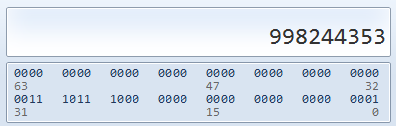void NTT(int* a,int len,int opt){
for(int i=0;i<len;i++){
if(i<rev[i]){
std::swap(a[i],a[rev[i]]);
}
}
for(int i=1;i<len;i<<=1){
int wn=Pow(G,(opt*((MOD-1)/(i<<1))+MOD-1)%(MOD-1),MOD);
int step=i<<1;
for(int j=0;j<len;j+=step){
int w=1;
for(int k=0;k<i;k++,w=(1ll*w*wn)%MOD){
int x=a[j+k];
int y=1ll*w*a[j+k+i]%MOD;
a[j+k]=(x+y)%MOD;
a[j+k+i]=(x-y+MOD)%MOD;
}
}
}
if(opt==-1){
int r=Pow(len,MOD-2,MOD);
for(int i=0;i<len;i++)
a[i]=1ll*a[i]*r%MOD;
}
}


(SSE是一个现代CPU指令集, 全称Streaming SIMD Extensions, 单指令多数据流扩展. 包含各种浮点运算指令/MMX整数运算强化指令/连续内存块传输优化指令, 可以理解为这个指令集就是专门为各种现代化图形/科学计算设计的)

• 如果你用 std::complex, 那么 FFT 一般都要慢于 NTT
• 如果你手写 Complex 而且没有开 -O2, 那么 NTT 一般快于 FFT
• 如果你手写 Complex 而且开了 -O2, 那么 FFT 一般快于 NTT

• $$p=1004535809=479\times2^{21}+1$$, $$g=3$$
• $$p=469762049=7\times2^{26}+1$$, $$g=3$$ (这个NTT长度更长, 但是数值有些偏小)

$$3$$ $$1$$ $$1$$ $$2$$
$$5$$ $$1$$ $$2$$ $$2$$
$$17$$ $$1$$ $$4$$ $$3$$
$$97$$ $$3$$ $$5$$ $$5$$
$$193$$ $$3$$ $$6$$ $$5$$
$$257$$ $$1$$ $$8$$ $$3$$
$$7681$$ $$15$$ $$9$$ $$17$$
$$12289$$ $$3$$ $$12$$ $$11$$
$$40961$$ $$5$$ $$13$$ $$3$$
$$65537$$ $$1$$ $$16$$ $$3$$
$$786433$$ $$3$$ $$18$$ $$10$$
$$5767169$$ $$11$$ $$19$$ $$3$$
$$7340033$$ $$7$$ $$20$$ $$3$$
$$23068673$$ $$11$$ $$21$$ $$3$$
$$104857601$$ $$25$$ $$22$$ $$3$$
$$167772161$$ $$5$$ $$25$$ $$3$$
$$469762049$$ $$7$$ $$26$$ $$3$$
$$1004535809$$ $$479$$ $$21$$ $$3$$
$$2013265921$$ $$15$$ $$27$$ $$31$$
$$2281701377$$ $$17$$ $$27$$ $$3$$
$$3221225473$$ $$3$$ $$30$$ $$5$$
$$75161927681$$ $$35$$ $$31$$ $$3$$
$$77309411329$$ $$9$$ $$33$$ $$7$$
$$206158430209$$ $$3$$ $$36$$ $$22$$
$$2061584302081$$ $$15$$ $$37$$ $$7$$
$$2748779069441$$ $$5$$ $$39$$ $$3$$
$$6597069766657$$ $$3$$ $$41$$ $$5$$
$$39582418599937$$ $$9$$ $$42$$ $$5$$
$$79164837199873$$ $$9$$ $$43$$ $$5$$
$$263882790666241$$ $$15$$ $$44$$ $$7$$
$$1231453023109121$$ $$35$$ $$45$$ $$3$$
$$1337006139375617$$ $$19$$ $$46$$ $$3$$
$$3799912185593857$$ $$27$$ $$47$$ $$5$$
$$4222124650659841$$ $$15$$ $$48$$ $$19$$
$$7881299347898369$$ $$7$$ $$50$$ $$6$$
$$31525197391593473$$ $$7$$ $$52$$ $$3$$
$$180143985094819841$$ $$5$$ $$55$$ $$6$$
$$1945555039024054273$$ $$27$$ $$56$$ $$5$$
$$4179340454199820289$$ $$29$$ $$57$$ $$3$$

### 任意模数 NTT

$\begin{cases} x\equiv a_1\pmod {p_1} \\ x\equiv a_2\pmod {p_2} \\ x\equiv a_3\pmod {p_3} \end{cases}$

\left \{ \begin{aligned} x &\equiv A \pmod M \\ x &\equiv a_3 \pmod {p_3} \\ \end{aligned} \right.

$x=kM+A$

$$k$$ 要满足:

$kM+A\equiv a_3 \pmod{p_3}$

$k \equiv (a_3 - A)M^{-1} \pmod {p_3}$

$x=kM+A \pmod{p}$

LL Mul(LL a,LL b,LL P){
a=(a%P+P)%P,b=(b%P+P)%P;
return ((a*b-(LL)((long double)a/P*b+1e-6)*P)%P+P)%P;
}


## 卷积状物体与分治 FFT

$f(x)=\sum_{i=1}^xf(x-i)g(i)$

(注意求和下指标是从 $$1$$ 开始的)

$$O(n^2)$$ 的算法非常显然, 根据定义式从小到大枚举 $$x$$ 就可以计算了.

$F(x)=\sum_{i=1}^xf(i)g(x-i)$

void cdq(int l,int r){
if(l==r) return;
int mid=l+r>>1;
cdq(l,mid);

int up=r-l-1;
//	for(lg=0,sze=1;sze<=(up<<1);sze<<=1)++lg;--lg;
for(lg=0,sze=1;sze<=up;sze<<=1)++lg;--lg;//其实只用的到区间长度，实际却有两倍，所以保险用两倍。
for(int i=0;i<sze;i++) R[i]=(R[i>>1]>>1)|((i&1)<<lg),A[i]=B[i]=0;
//	memset(A,0,sizeof(A));memset(B,0,sizeof(B));
//	fill(A,A+sze,0);fill(B,B+sze,0);//这两种方法赋值fill会比memset快一些（因为fill确定了大小）
for(int i=l;i<=mid;i++) A[i-l]=f[i];
for(int i=1;i<=r-l;i++) B[i-1]=g[i];
calc(A,B,sze);

for(int i=mid+1;i<=r;i++) f[i]=(f[i]+A[i-l-1]%mod)%mod;
cdq(mid+1,r);
}


calc 函数是计算 AB 的卷积并把结果放在 A 里.

## FWT 与位运算卷积

$c_k=\sum_{i\circ j=k}a_i b_j$

### FWT 与 $$\text{or}$$ 卷积

#### 构造变换 $$T$$

$$x\ \text{or}\ y=z$$ , 那么 $$x$$$$y$$ 一定满足它们的二进制代表的集合都是 $$z$$ 的子集.

$T(A)_i=\sum_{j\subseteq i}A_j$

$T(A*B)_i=\sum_{j\subseteq i}\sum_{a\cup b=j}A_aB_b$

$(T(A)\cdot T(B))_i=\left (\sum_{a\subseteq i}A_a \right )\left(\sum_{b\subseteq i}B_b\right)$

$\sum_{(a\cup b)\subseteq i}A_aB_b$

#### 计算变换 $$T$$

• $$A_0$$ 相当于是第 $$k$$ 位填 $$0$$ (不选), 那么子集肯定还是只有 $$T(A_0)$$ 里的值.
• $$A_1$$ 相当于是第 $$k$$ 位填 $$1$$ (选中), 那么子集除了 $$T(A_1)$$ 里的值, 还有 $$T(A_0)$$ 里的值.

$$A=(B,C)$$ 表示把 $$B$$$$C$$ 首尾相接得到的数列赋给 $$A$$, 那么:

$T(A)=(T(A_0),T(A_1)+T(A_0))$

#### 计算逆变换 $$T^{-1}$$

$T^{-1}(A)=(T^{-1}(A_0),T^{-1}(A_1)-T^{-1}(A_0))$

#### 迭代实现

void FWT(LL *a,int opt){
for(int i=2;i<=N;i<<=1)
for(int p=i>>1,j=0;j<N;j+=i)
for(int k=j;k<j+p;++k)
a[k+p]+=a[k]*opt;
}


### FWT 与 $$\text{and}$$ 卷积

#### 构造变换 $$T$$

$$x\ \text{and}\ y=z$$, 那么 $$x$$$$y$$ 一定都满足它们的二进制代表的集合都是 $$z$$ 的超集.

$T(A)_i=\sum_{j\supseteq i}A_j$

#### 计算变换 $$T$$

$T(A)=(T(A_0)+T(A_1),T(A_1))$

• $$A_0$$ 相当于是第 $$k$$ 位填 $$0$$ (不选), 那么超集除了 $$T(A_0)$$ 里的值, 还有 $$T(A_1)$$ 里的值.
• $$A_1$$ 相当于是第 $$k$$ 位填 $$1$$ (选中), 那么超集肯定还是只有 $$T(A_1)$$ 里的值.

#### 计算逆变换 $$T^{-1}$$

$T^{-1}(A)=(T^{-1}(A_0)-T^{-1}(A_1),T^{-1}(A_1))$

#### 迭代实现

void FWT(LL *a,int opt){
for(int i=2;i<=N;i<<=1)
for(int p=i>>1,j=0;j<N;j+=i)
for(int k=j;k<j+p;++k)
a[k]+=a[k+p]*opt;
}


### FWT 与 $$\text{xor}$$ 卷积

#### 构造变换 $$T$$

$$\text{and}$$$$\text{or}$$ 卷积中, 我们利用了一些集合操作成功地构造出了一个卷积性变换, 然而 $$\text{xor}$$ 可就没有这样优秀的性质了...

$$d(x)$$ 表示 $$x$$ 的二进制表示中 $$1$$ 的个数的奇偶性, 则我们有:

$d(i\,\text{and}\,k)\,\text{xor}\,d(j\,\text{and}\,k)=d((i\,\text{xor}\,j)\,\text{and}\,k)$

$T(A)_i=\sum_{d(i\,\text{and}\,j)=0}A_j-\sum_{d(i\,\text{and}\,j)=1}A_j$

$T(A*B)_i=\sum_{d(i\,\text{and}\,j)=0}\sum_{a\,\text{xor}\,b=j}A_aB_b-\sum_{d(i\,\text{and}\,j)=1}\sum_{a\,\text{xor}\,b=j}A_aB_b \\$

\begin{aligned} (T(A)\cdot T(B))_i&= \left( \sum_{d(i\,\text{and}\,j)=0}A_j-\sum_{d(i\,\text{and}\,j)=1}A_j \right) \left( \sum_{d(i\,\text{and}\,j)=0}B_j-\sum_{d(i\,\text{and}\,j)=1}B_j \right) \\ &= \left( \left( \sum_{d(i\,\text{and}\,j)=0}A_j \right) \left( \sum_{d(i\,\text{and}\,j)=0}B_j \right) \right)+ \left( \left( \sum_{d(i\,\text{and}\,j)=1}A_j \right) \left( \sum_{d(i\,\text{and}\,j)=1}B_j \right) \right)- \left( \left( \sum_{d(i\,\text{and}\,j)=0}A_j \right) \left( \sum_{d(i\,\text{and}\,j)=1}B_j \right) \right)- \left( \left( \sum_{d(i\,\text{and}\,j)=1}A_j \right) \left( \sum_{d(i\,\text{and}\,j)=0}B_j \right) \right)\\ &= \left( \left( \sum_{d(i\,\text{and}\,j)=0}A_j \right) \left( \sum_{d(i\,\text{and}\,j)=0}B_j \right)+ \left( \sum_{d(i\,\text{and}\,j)=1}A_j \right) \left( \sum_{d(i\,\text{and}\,j)=1}B_j \right) \right)- \left( \left( \sum_{d(i\,\text{and}\,j)=0}A_j \right) \left( \sum_{d(i\,\text{and}\,j)=1}B_j \right)+ \left( \sum_{d(i\,\text{and}\,j)=1}A_j \right) \left( \sum_{d(i\,\text{and}\,j)=0}B_j \right) \right)\\ &= \left( \sum_{d(i\,\text{and}\,a)\,\text{xor}\,d(i\,\text{and}\,b)=0}A_aB_b \right)- \left( \sum_{d(i\,\text{and}\,a)\,\text{xor}\,d(i\,\text{and}\,b)=1}A_aB_b \right) \end{aligned}

$\sum_{d(i\,\text{and}\,a)\,\text{xor}\,d(i\,\text{and}\,b)=0}A_aB_b =\sum_{d(i\,\text{and}\,(a\,\text{xor}\,b))=0} A_aB_b =\sum_{d(i\,\text{and}\,j)=0}\sum_{a\,\text{xor}\,b=j}A_aB_b \\ \sum_{d(i\,\text{and}\,a)\,\text{xor}\,d(i\,\text{and}\,b)=1}A_aB_b =\sum_{d(i\,\text{and}\,(a\,\text{xor}\,b))=1} A_aB_b =\sum_{d(i\,\text{and}\,j)=1}\sum_{a\,\text{xor}\,b=j}A_aB_b$

#### 计算变换 $$T$$

(不过还好它们没有变成环)

• 当最高位是 $$0$$ 的时候, $$\text{and}$$ 运算的结果并不产生影响, 所以我们直接把 $$T(A_0)$$$$T(A_1)$$ 的相对位置加起来就可以了.

• 当最高位是 $$1$$ 的时候, $$\text{and}$$ 运算的结果可能会产生区别. 左半部分因为下标最高位是 $$0$$ 于是直接产生贡献, 右半部分下标最最高位是 $$1$$, 于是多了一个翻转奇偶性的贡献, 翻转奇偶性之后根据定义式, 两个求和号的内容互换, 相当于交换符号, 于是我们把它取反加在对应位置.

$T(A)=(T(A_0)+T(A_1),T(A_0)-T(A_1))$

#### 计算逆变换 $$T^{-1}$$

$T(A)=(T(A_0)+T(A_1),T(A_0)-T(A_1))$

$T^{-1}(A)=\left(\frac{T^{-1}(A_0)+T^{-1}(A_1)}2,\frac{T^{-1}(A_0)-T^{-1}(A_1)} 2\right)$

#### 迭代实现

void FWT(int *a,int opt){
for(int i=2;i<=N;i<<=1)
for(int p=i>>1,j=0;j<N;j+=i)
for(int k=j;k<j+p;++k)
{
int x=a[k],y=a[k+p];
a[k]=(x+y)%MOD;a[k+p]=(x-y+MOD)%MOD;
if(opt==-1)a[k]=1ll*a[k]*inv2%MOD,a[k+p]=1ll*a[k+p]*inv2%MOD;
}
}


inv2 是预处理的 $$2$$ 的逆元.

## FMT 与子集卷积

$F(S)=\sum_{T\subseteq S}f(T)g(S-T)$

$F(S)=\sum_{A\cup B=S,A\cap B=\varnothing}f(A)g(B)$

$F(S)=\sum_{T\subseteq S}f(T)$

inline void FMT(int *a,int len,int opt) {
for (int i = 1; i < len; i <<= 1)
for (int j = 0; j < len; ++j)
if (j & i)
a[j]+=a[j^i]*opt;
}


$F_k(S)=\sum_{T\subseteq S,|T|=k}f(T)$

\begin{align} P_k(S)&=\sum_{i+j=k}F_i(S)G_j(S) \\ &=\sum_{i+j=k} \left( \sum_{T\subseteq S,|T|=i}f(T) \right) \left( \sum_{T\subseteq S,|T|=j}g(T) \right) \\ &=\sum_{A\subseteq S,B\subseteq S,|A|+|B|=k}f(A)g(B) \\ &=\sum_{A\subseteq S,B\subseteq S}f(A)g(B)[|A|+|B|=k] \end{align}

\begin{align} p_k(S)&=\sum_{A\cup B=S} f(A)g(B)[|A|+|B|=k] \\ &=\sum_{A\cup B=S,|A|+|B|=k} f(A)g(B) \end{align}

## 卷积与字符串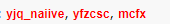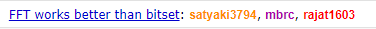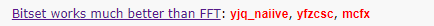### 卷积与回文序列

$f(x)=\sum_{i+j=x}[s_i=k][s_j=k]$

### 卷积与通配符

KMP/ST/SAM等等状态转移自动机肯定是不行的, 单次匹配倒是没问题. 但是单次匹配你为啥不直接暴力呢?

$$0$$ !

$M(x)=\sum_{i=0}^{\text{len}(P)-1}\left|S_{x+i}-P_i\right|\times S_{x+i}\times P_i$

\begin{align} M(x)&=\sum_{i=0}^{\text{len}(P)-1}(S_{x+i}-P_i)^2S_{x+i}P_i \\ &=\sum_{i=0}^{\text{len}(P)-1}(S_{x+i}^2-2S_{x+i}P_i+P_i^2)S_{x+i}P_i \\ &=\sum_{i=0}^{\text{len}(P)-1}S_{x+i}^3P_i-2S^2_{x+i}P^2_i+S_{x+i}P_i^3 \\ &= \left( \sum_{i=0}^{\text{len}(P)-1}S_{x+i}^3P_i \right) - 2\left( \sum_{i=0}^{\text{len}(P)-1}S^2_{x+i}P^2_i \right) + \left( \sum_{i=0}^{\text{len}(P)-1}S_{x+i}P_i^3 \right) \end{align}

$a-b=a+(-b)$

$P'_i=P_{\text{len}(P)-i}$

emmmmm...

(也就是说当你发现减法卷积的时候翻转其中一个就可以了)

### 一点优化?

(实际上我们本质上是多项式乘法再加法的形式, 所以按照点值表示的运算法则算就可以了, 总计 7 遍 FFT)

\begin{align} M(x)&=\sum_{k=0}^{\text{len}(P)-1}e^{\frac{i\pi(S_{x+k}-P_k)}\Sigma} \\ &=\sum_{k=0}^{\text{len}(P)-1}e^{\frac{i\pi S_{x+k}}\Sigma}e^{\frac{-i\pi P_k}\Sigma} \end{align}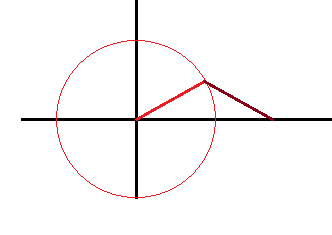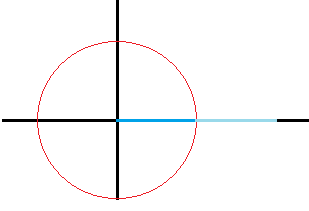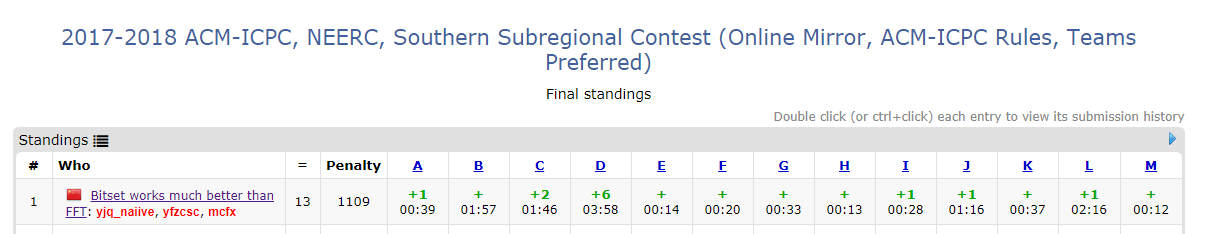(大雾

## 写在最后

posted @ 2018-12-14 17:34  rvalue  阅读(3987)  评论(9编辑  收藏  举报